# MSBSHSE Solutions For Class 9 Maths Part 2 Chapter 6 Circle

MSBSHSE Solutions For Class 9 Maths Part 2 Chapter 6 Circle provides answers to all Maharashtra Board exercises of Chapter 6. The clear diagrams given in our solutions help students better understand the concept of circle. This chapter deals with circles in a plane, congruent circles, concentric circles, circles intersecting in a point and circles intersecting in two points. BYJU’S provides precise solutions prepared by our experts. MSBSHSE Solutions For Class 9 Maths Part 2 Chapter 6 Circle can be downloaded in PDF format for free.

## Download the PDF of Maharashtra Solutions For Class 9 Maths Part 2 Chapter 6 Circle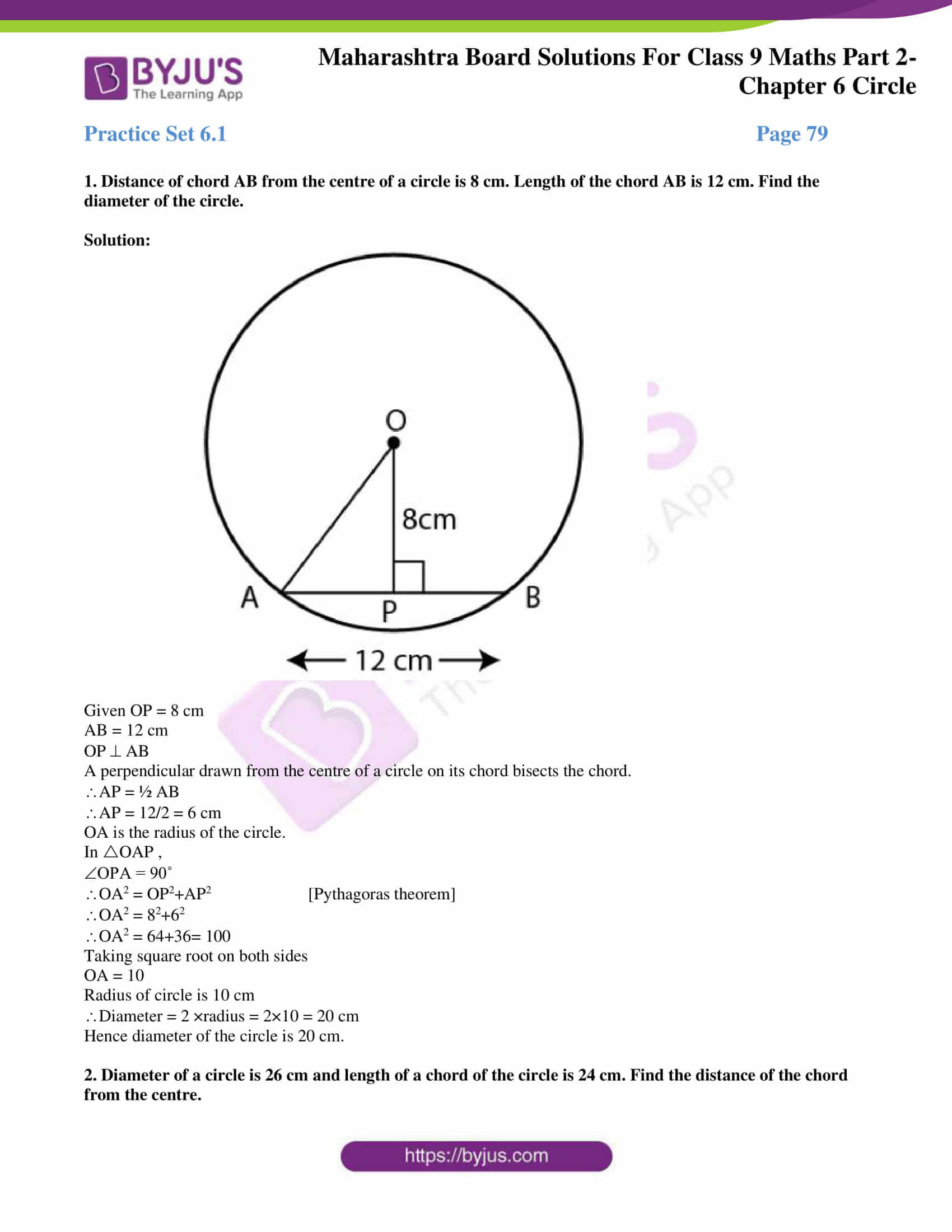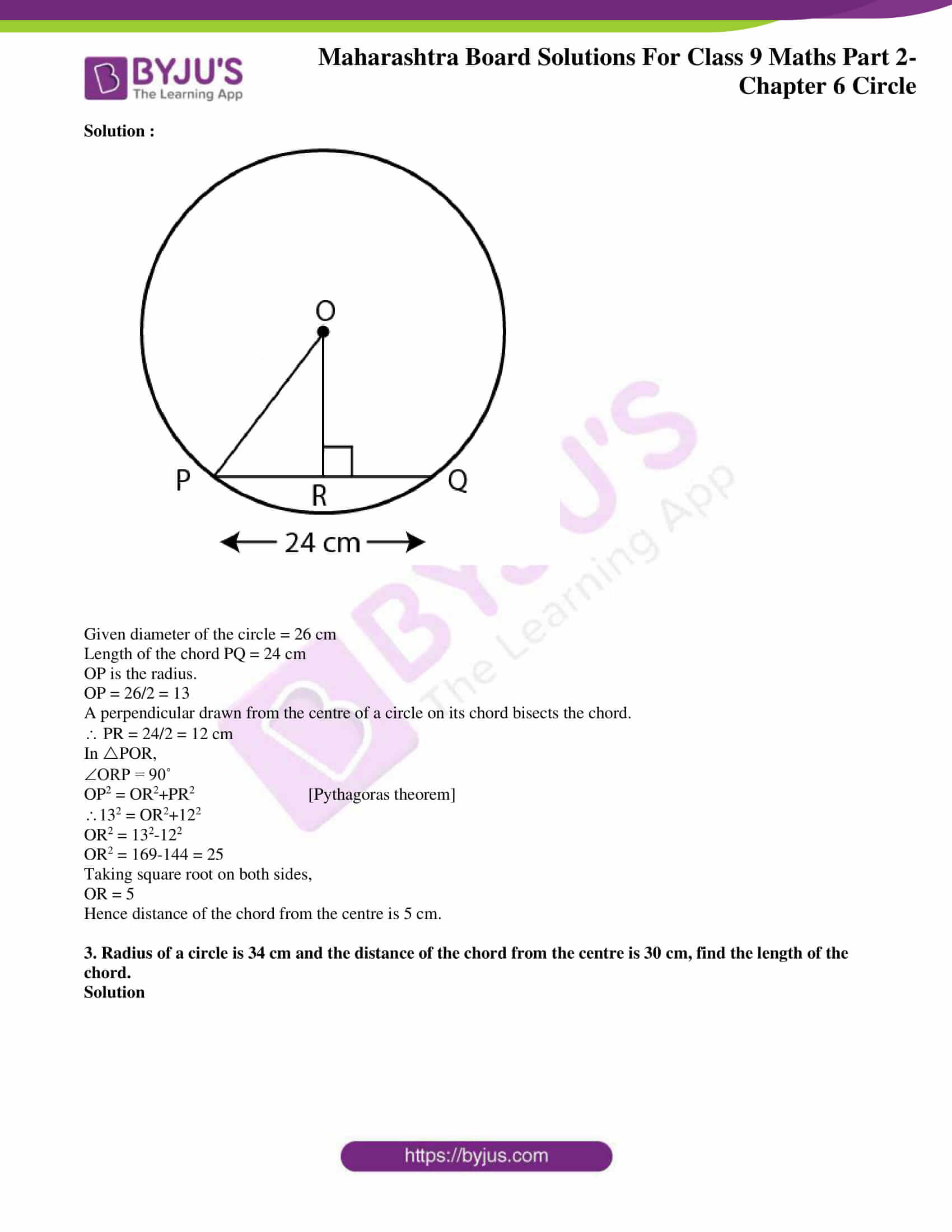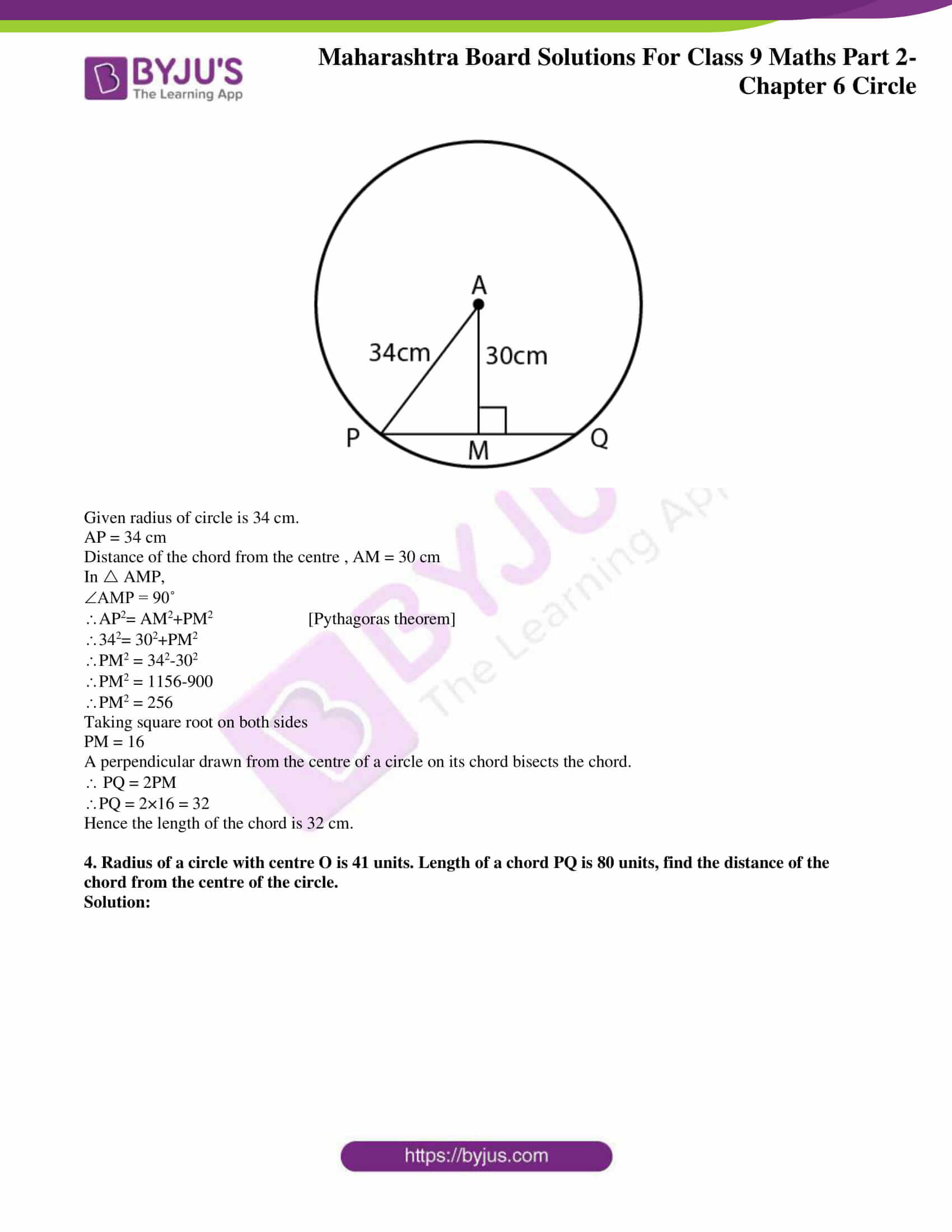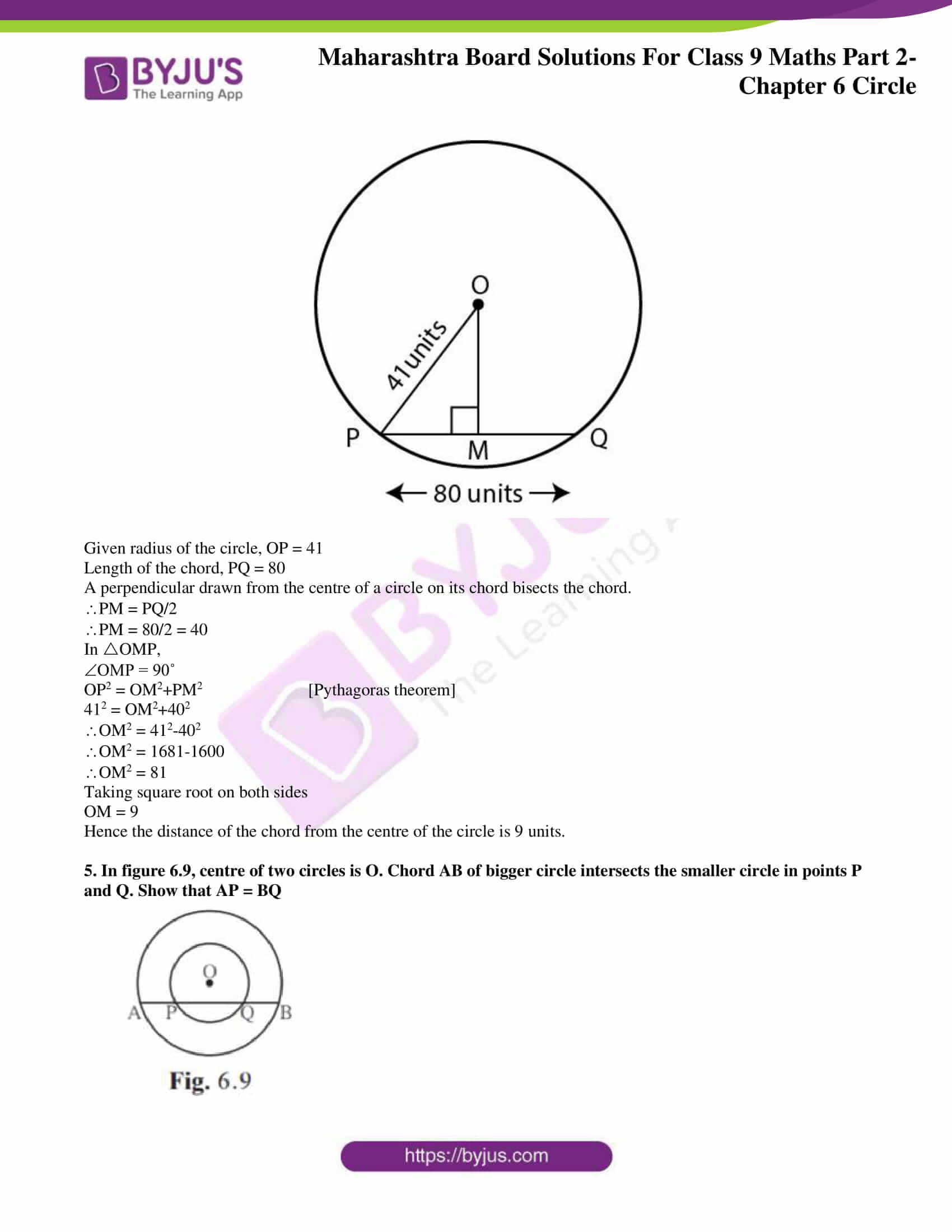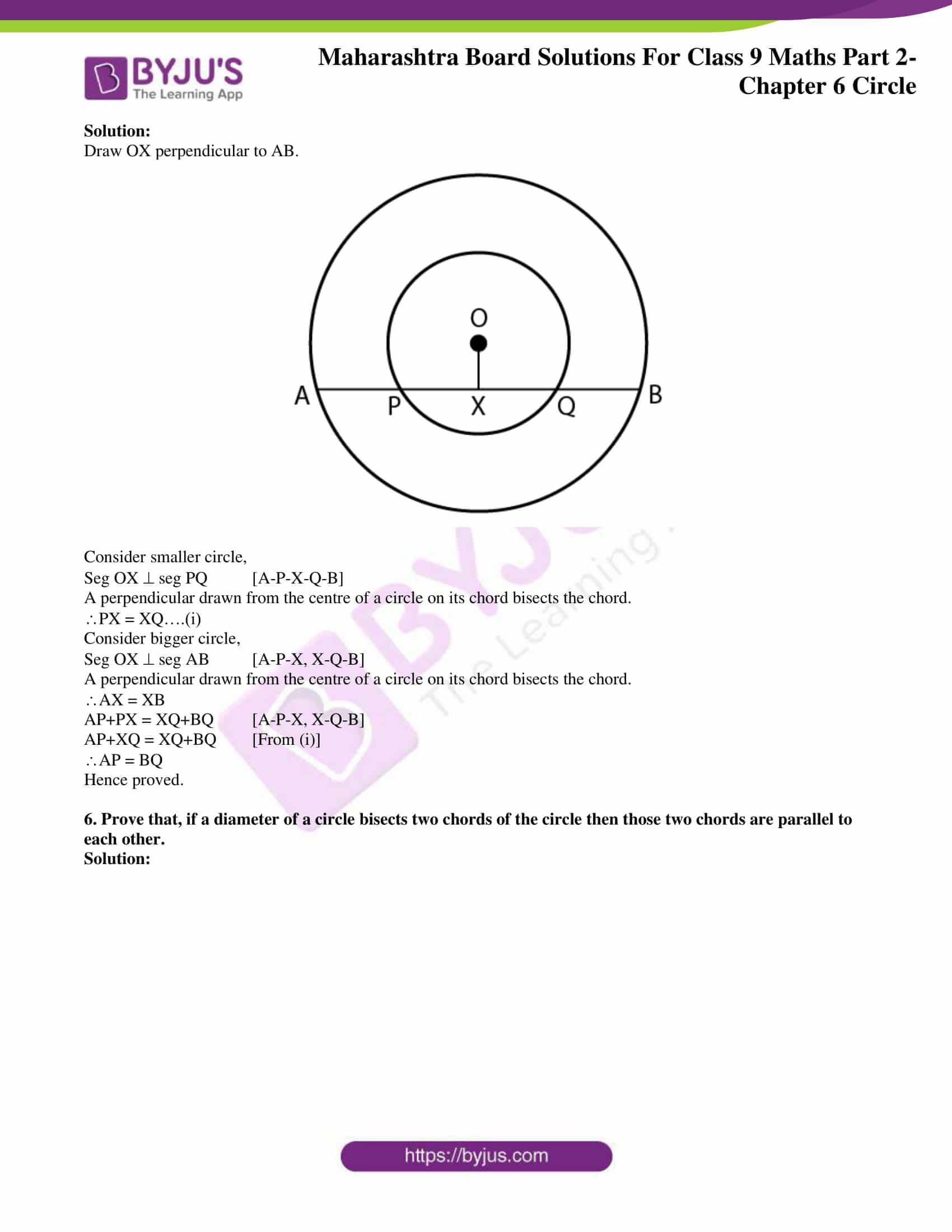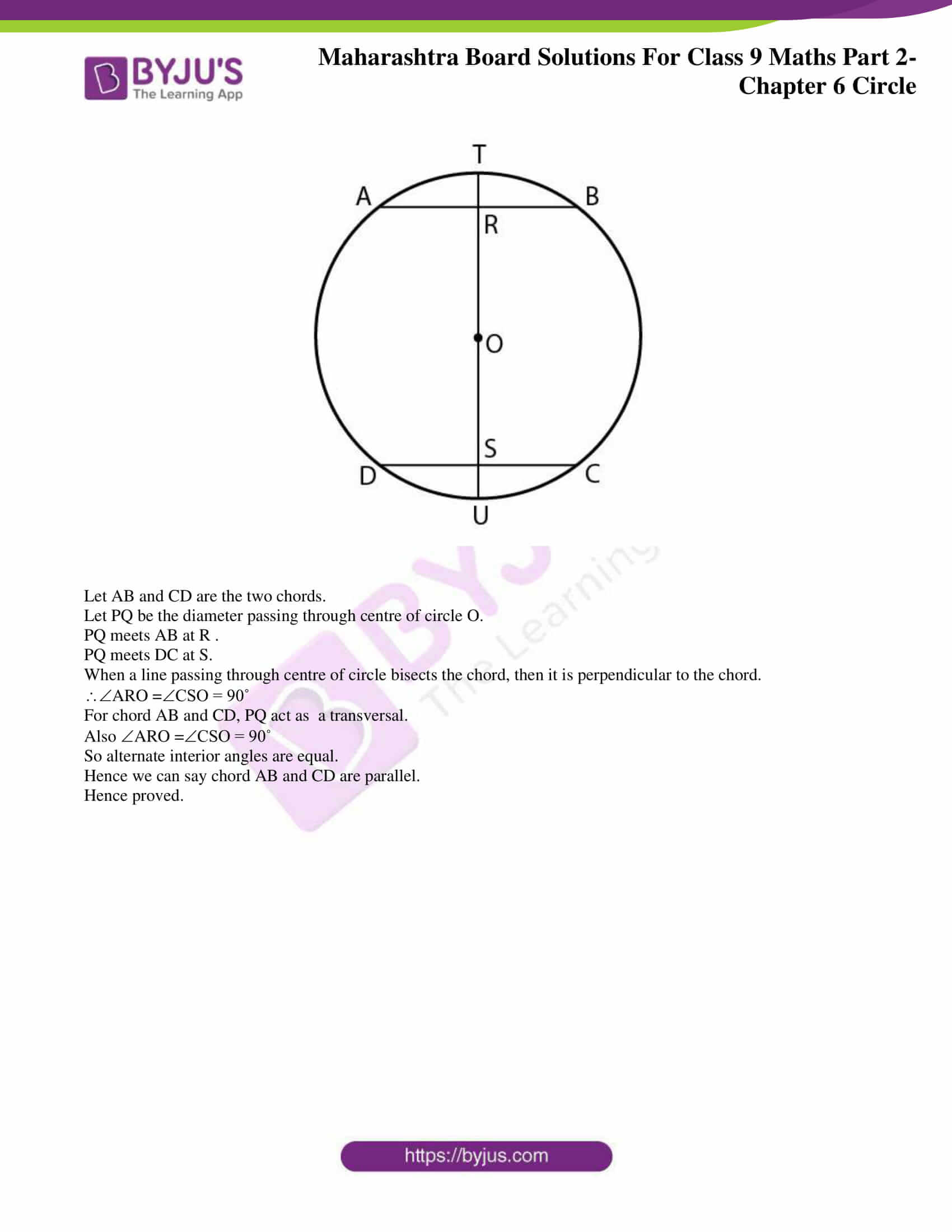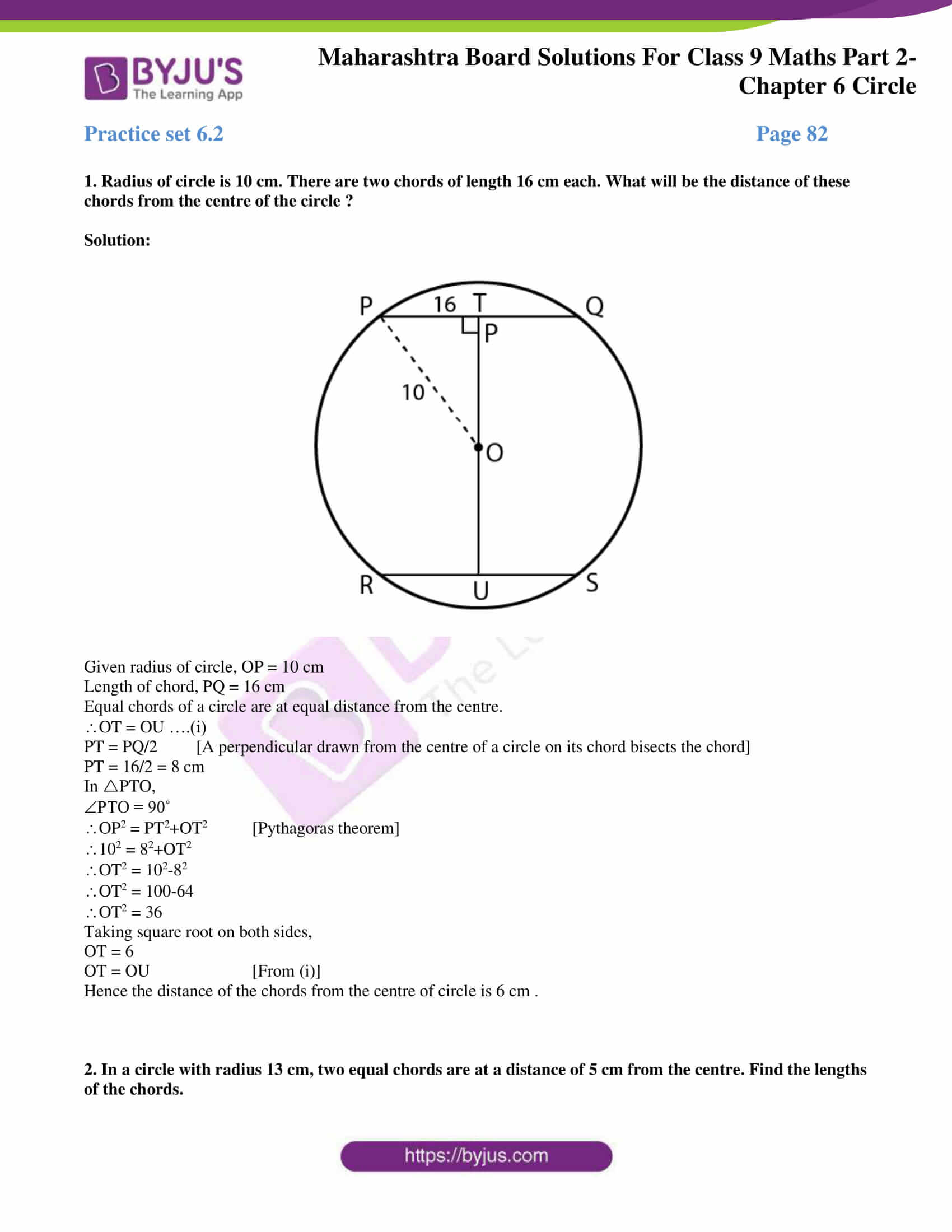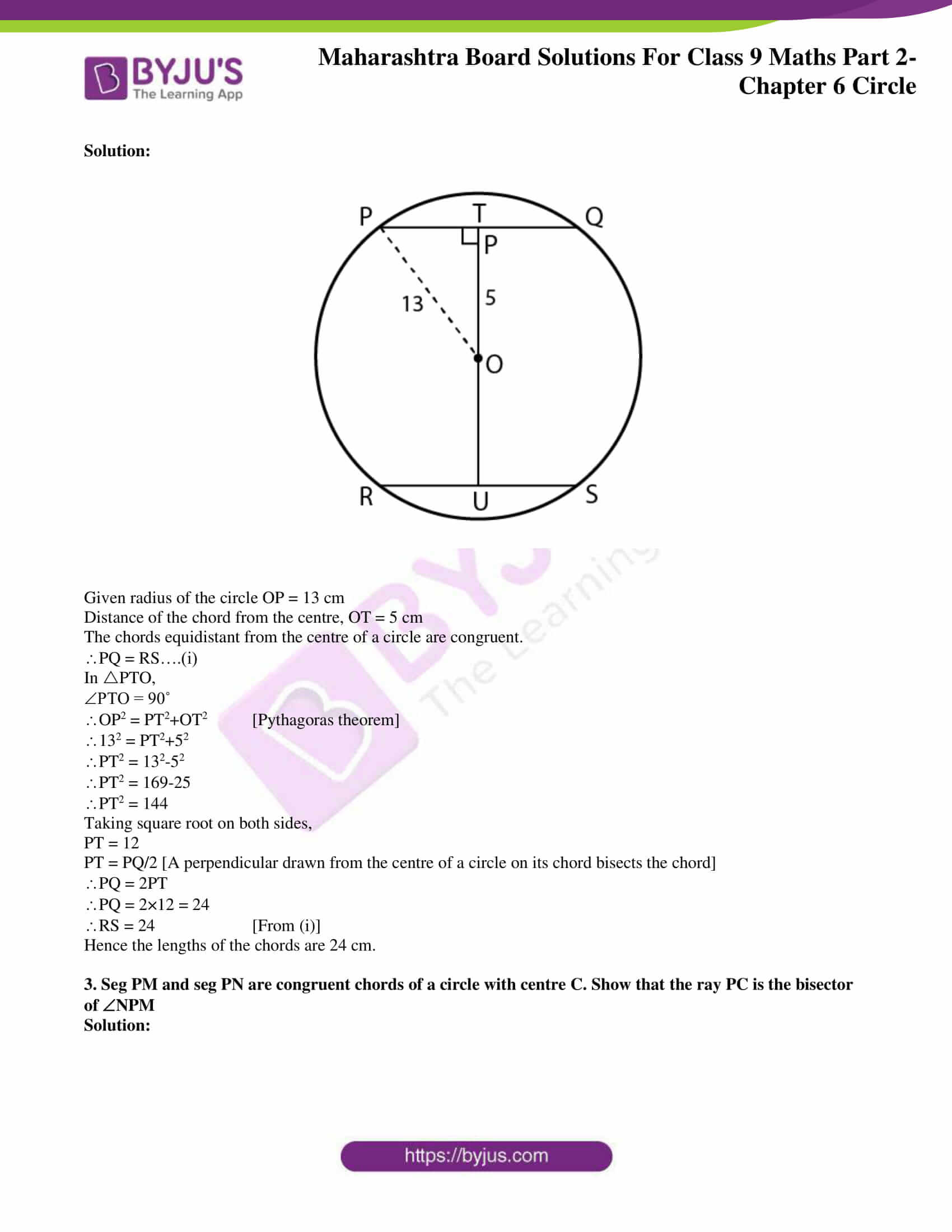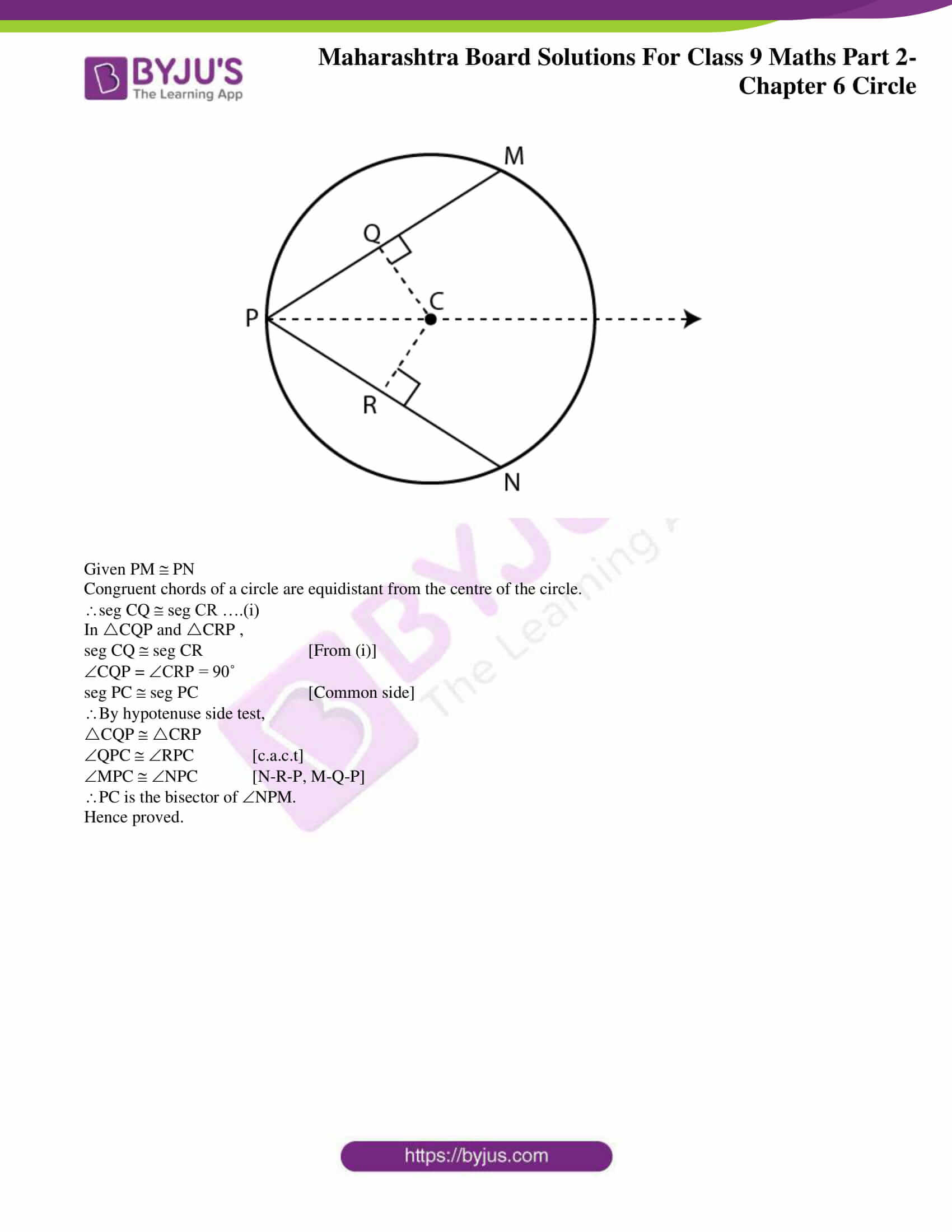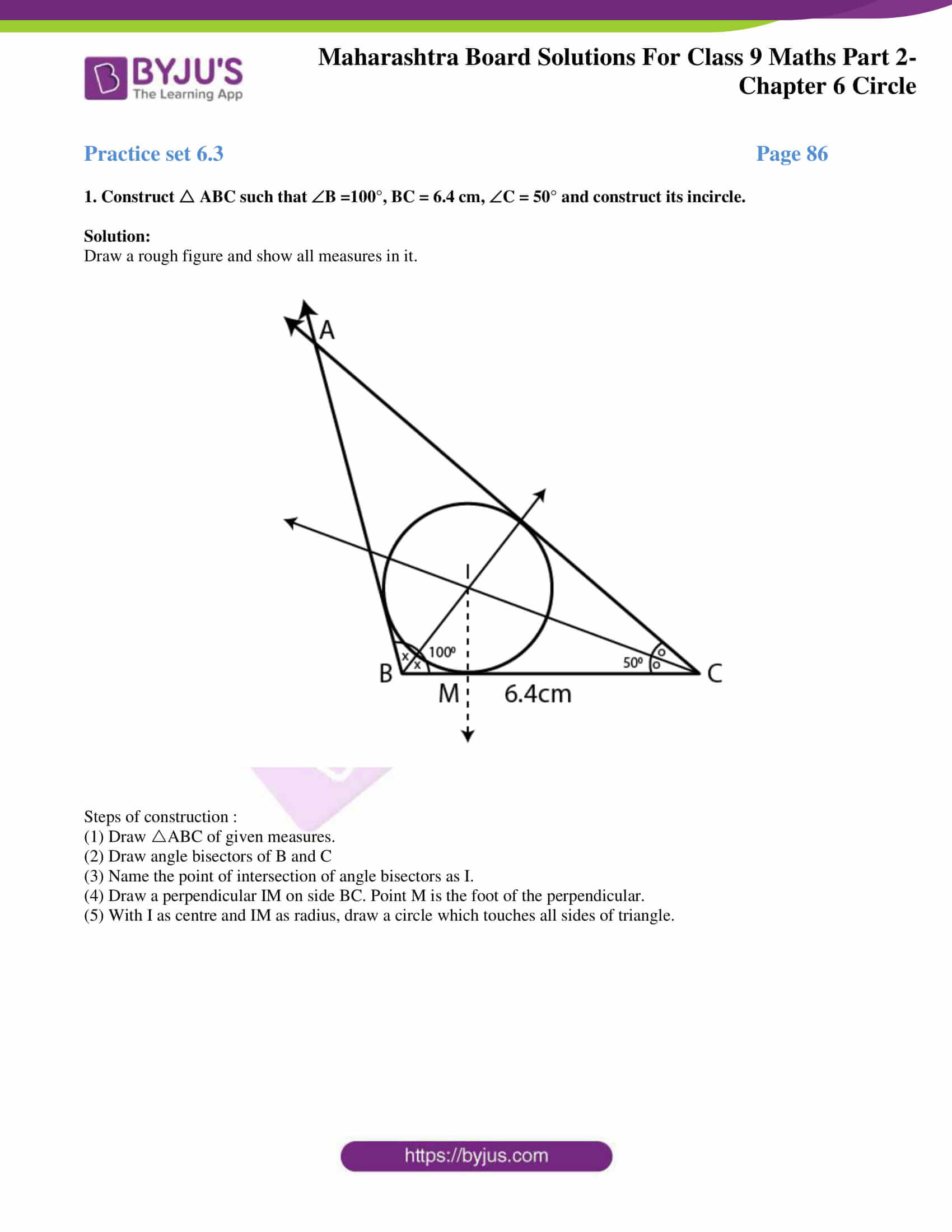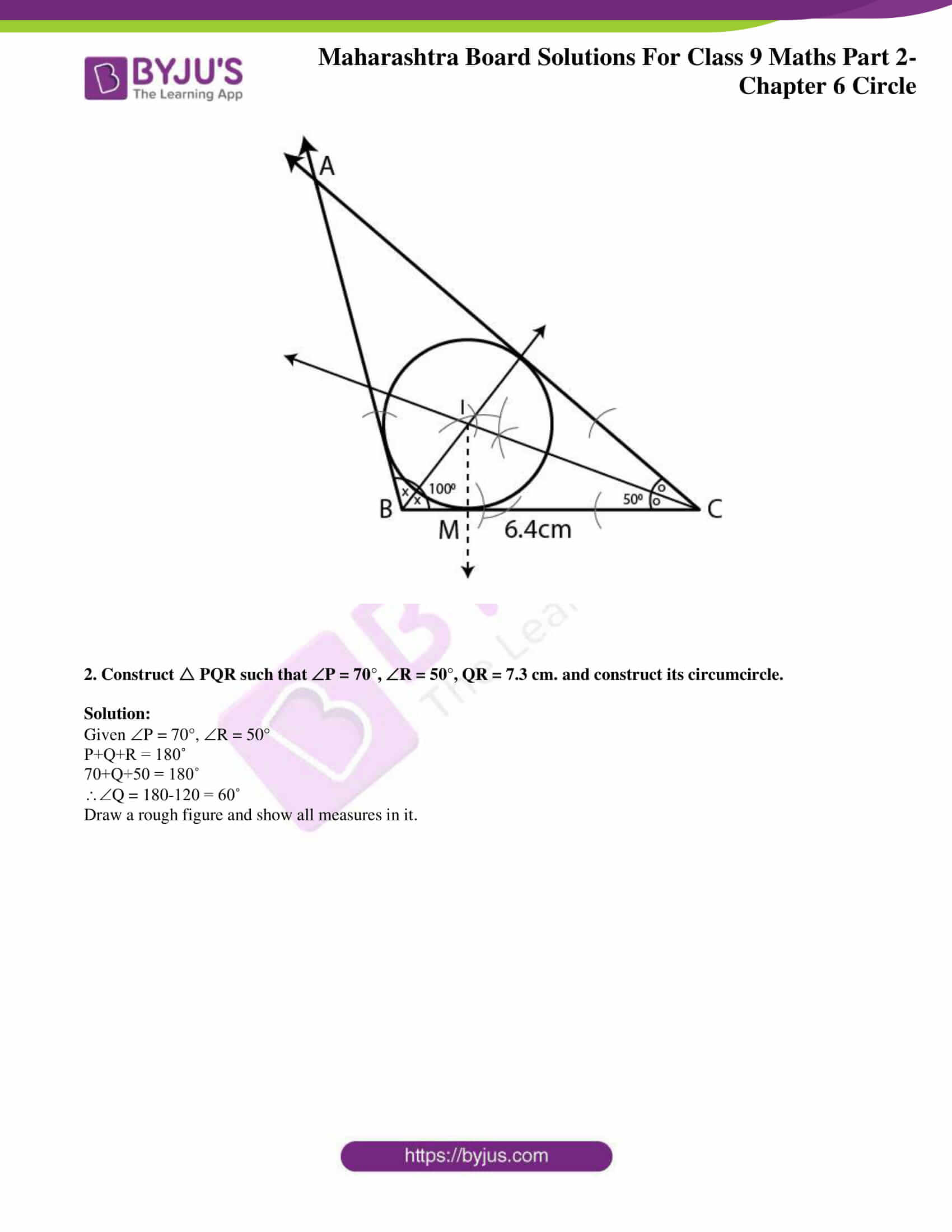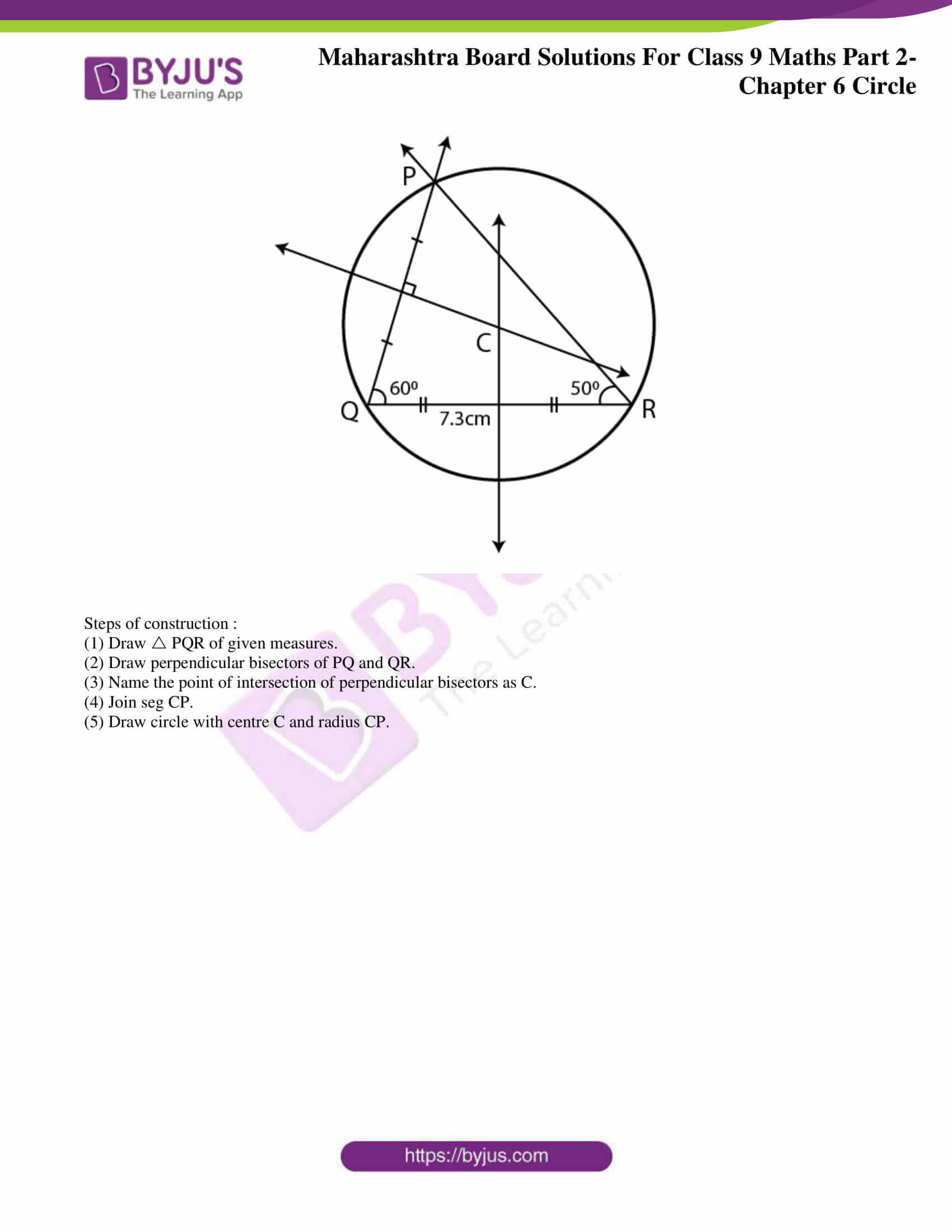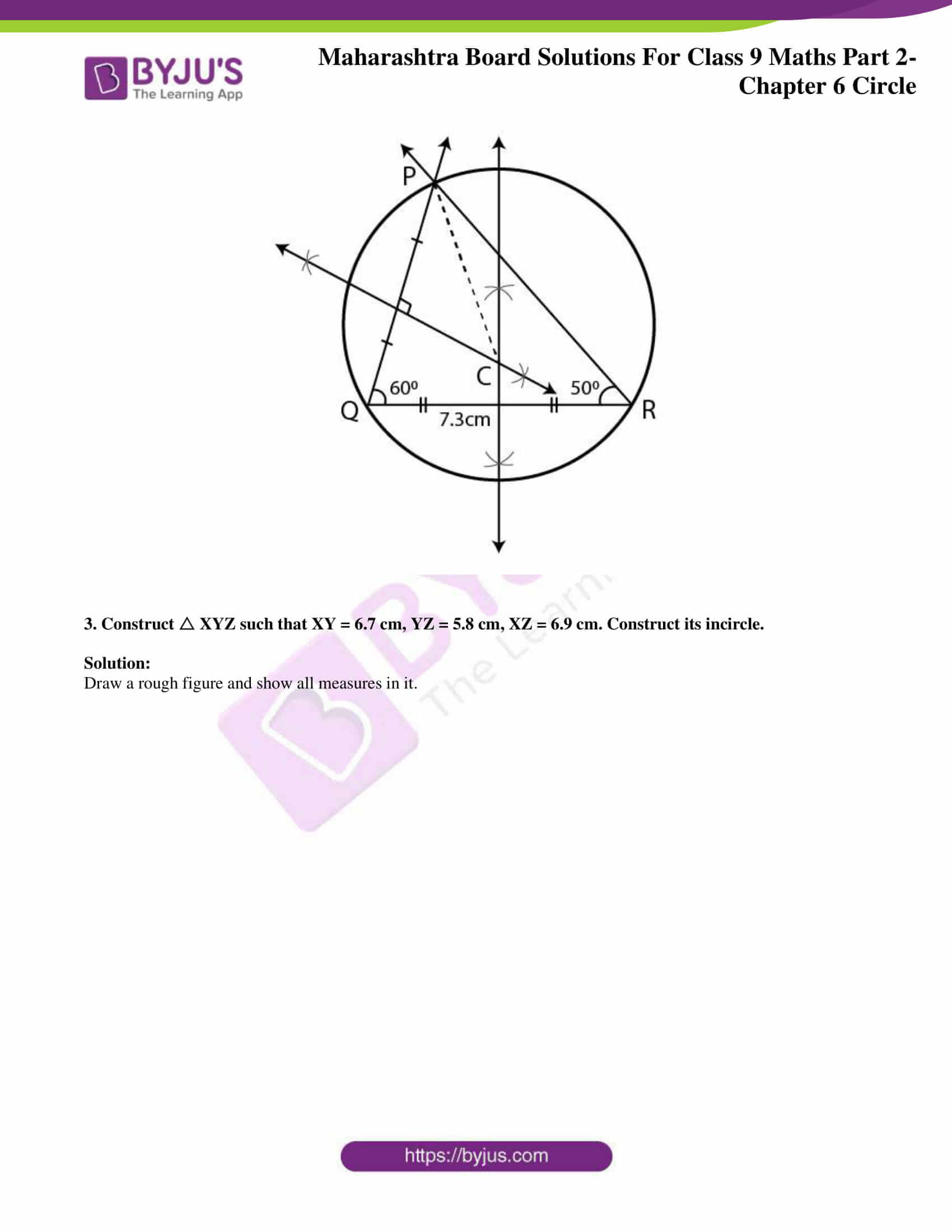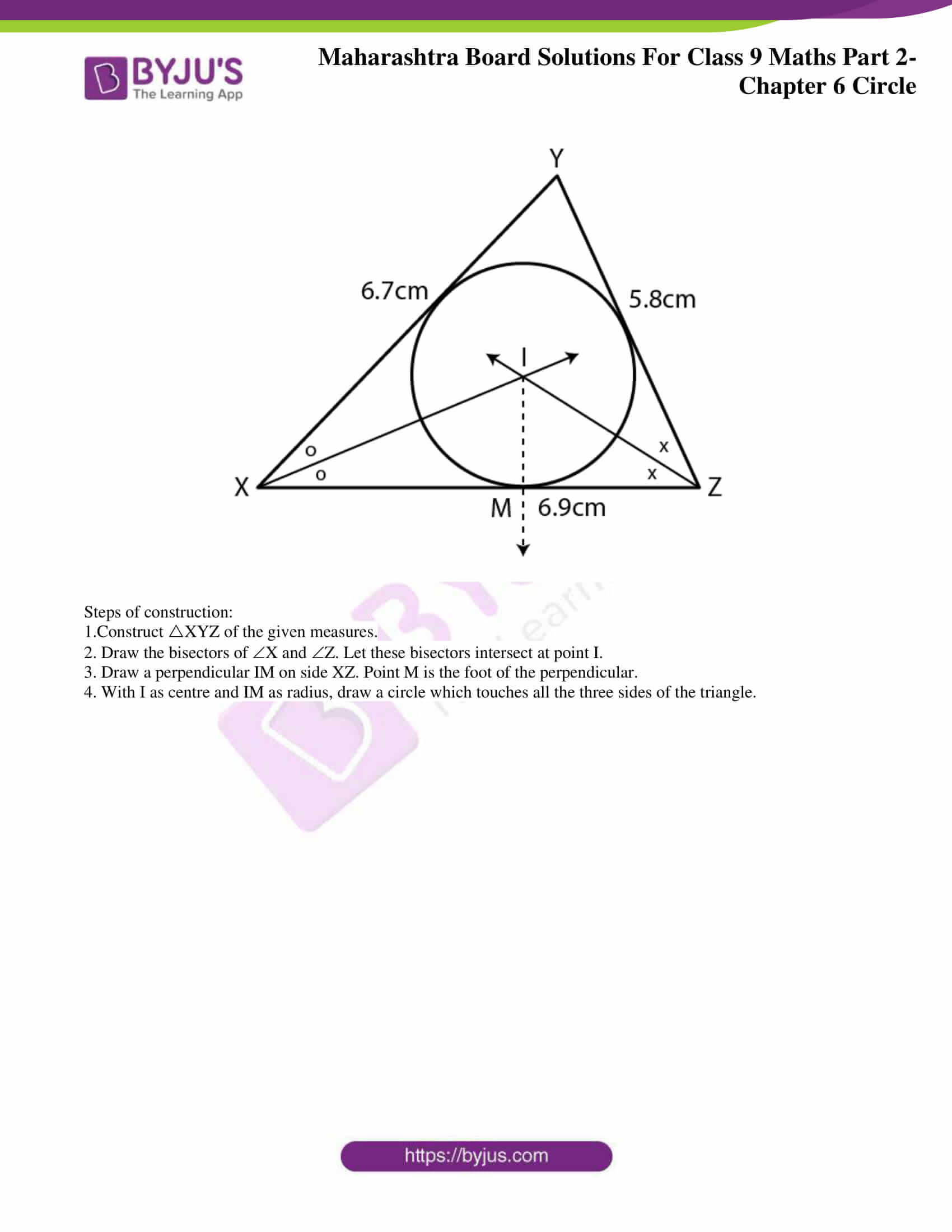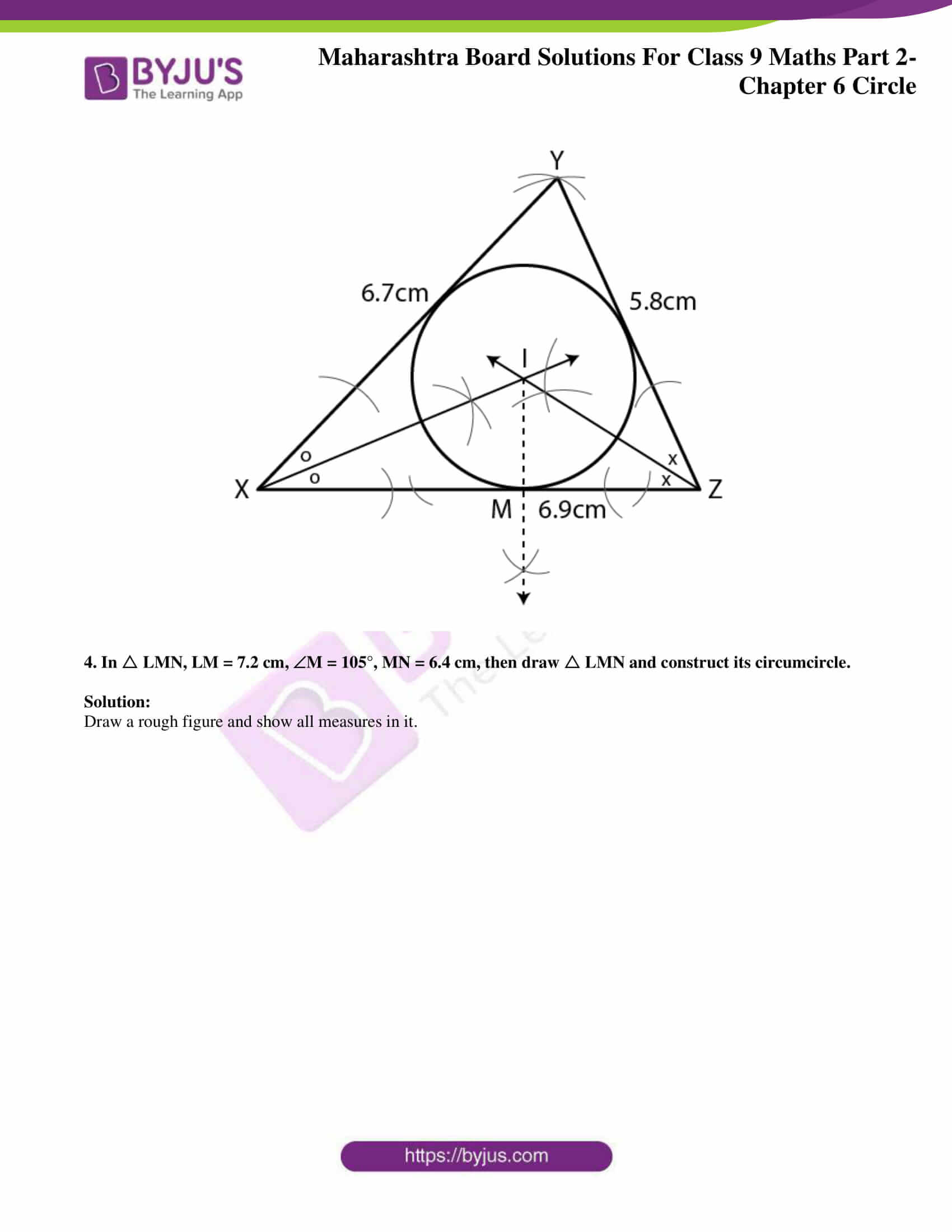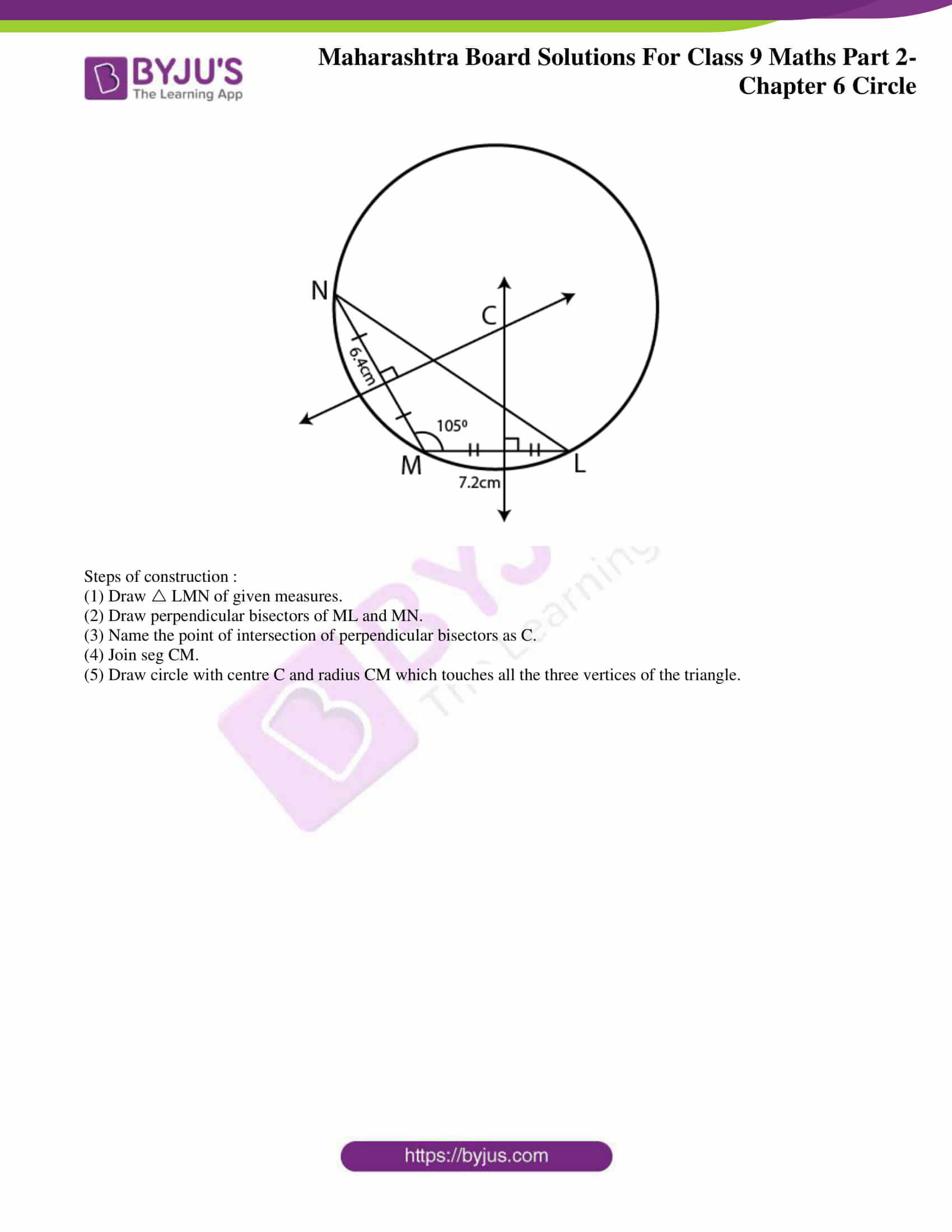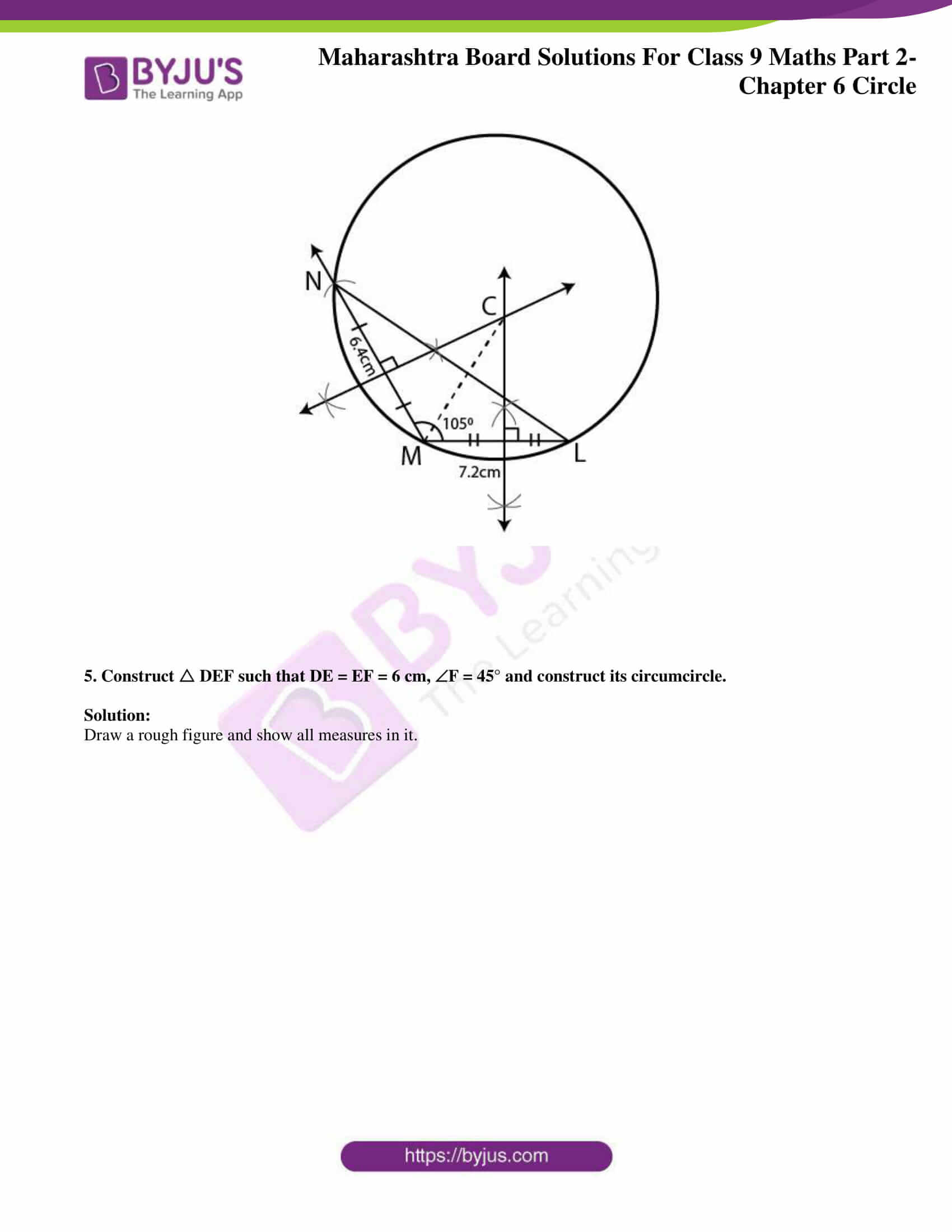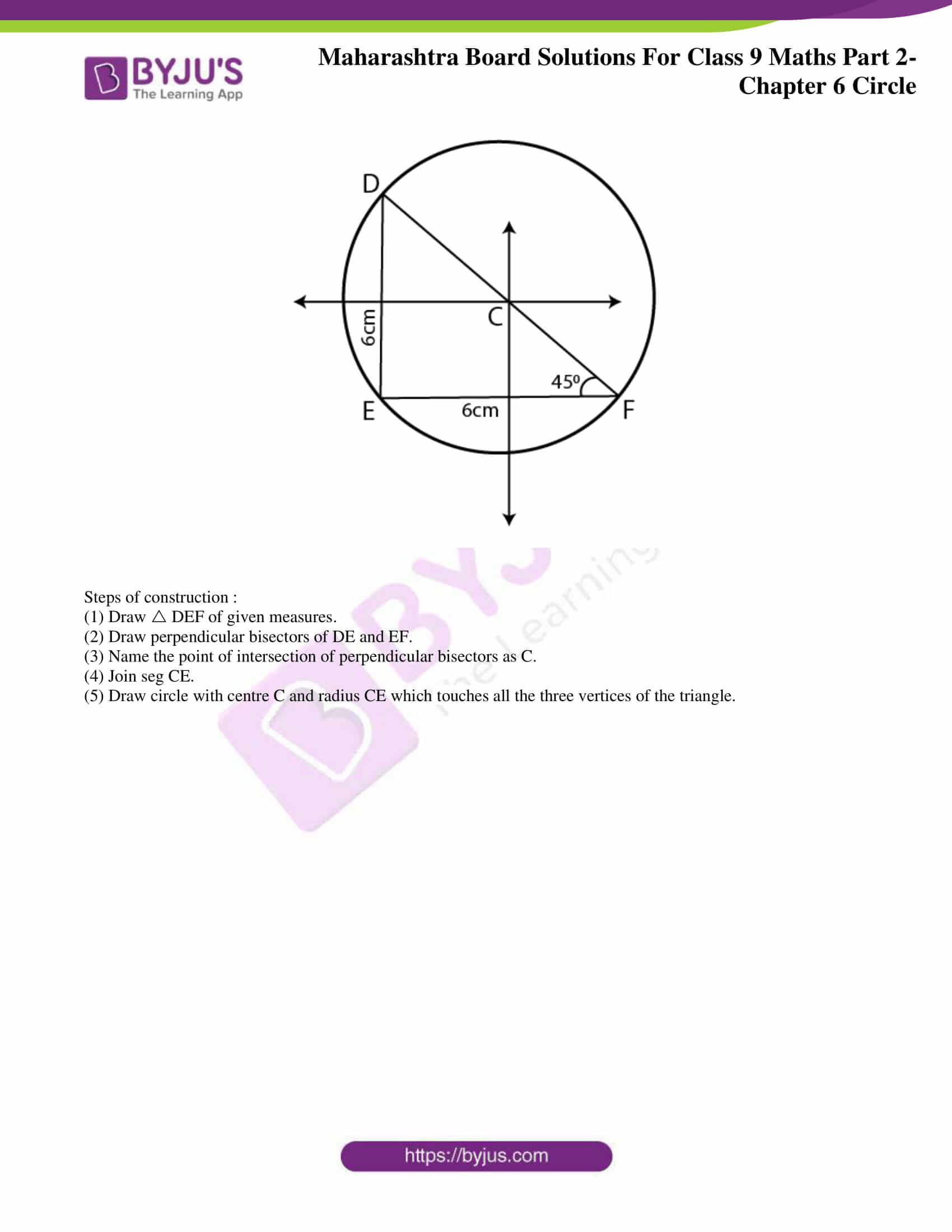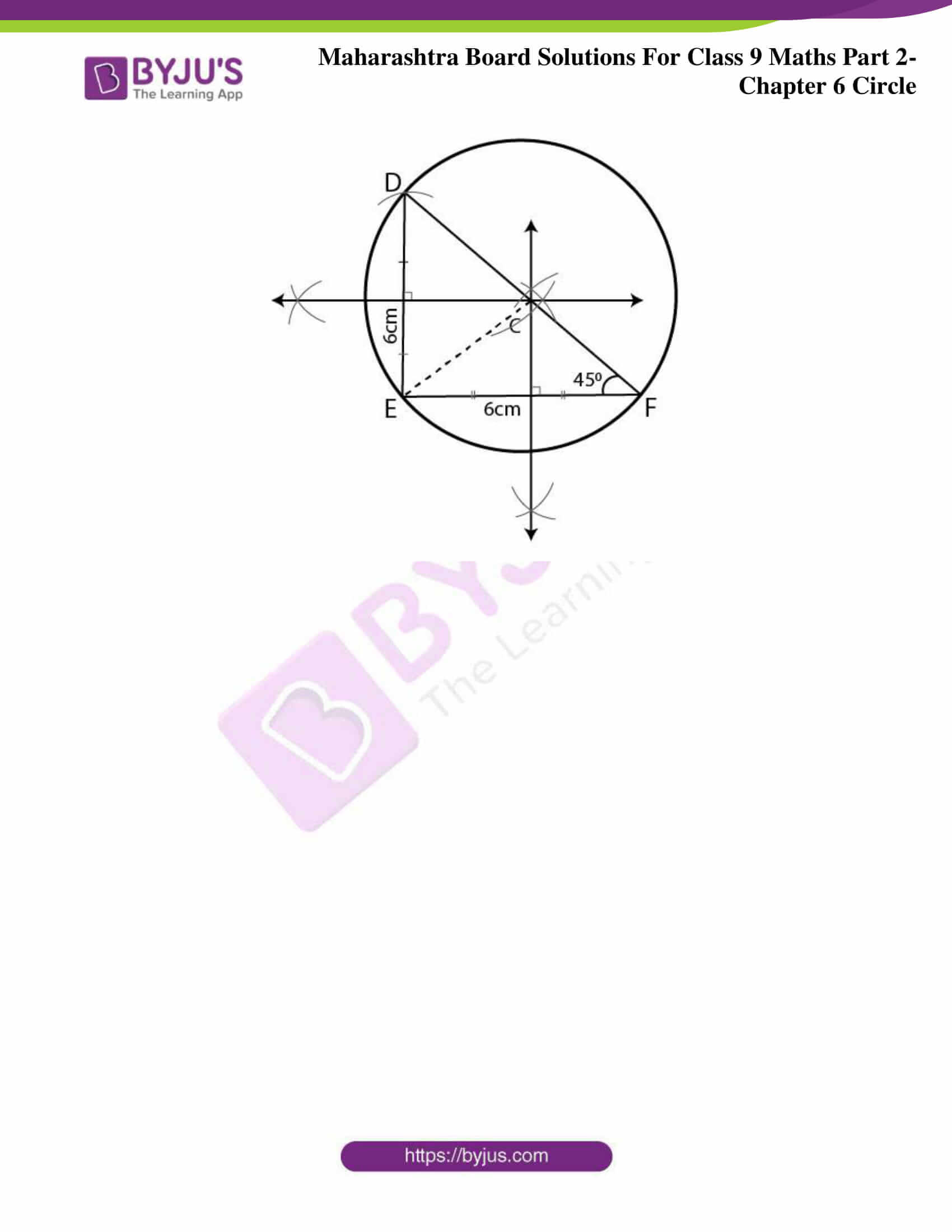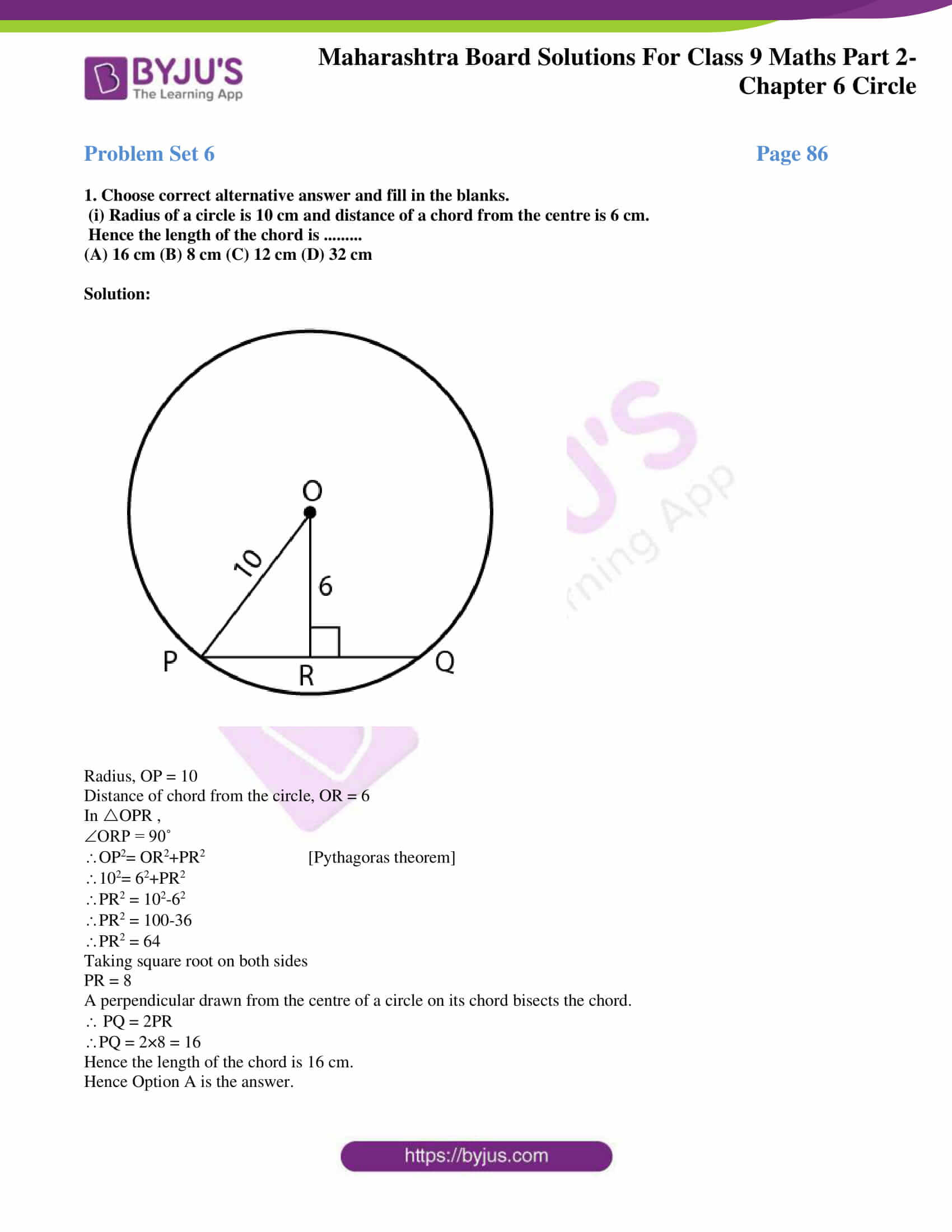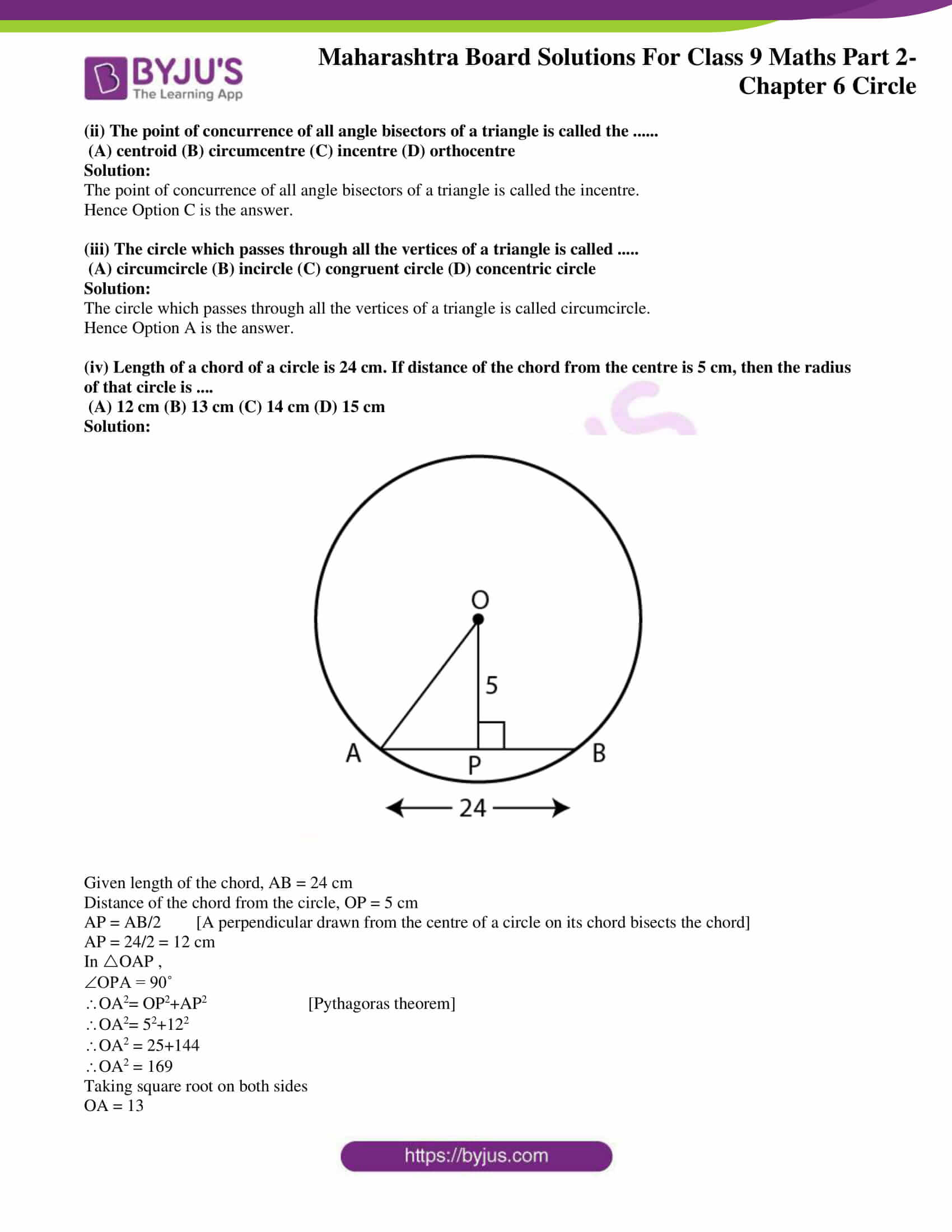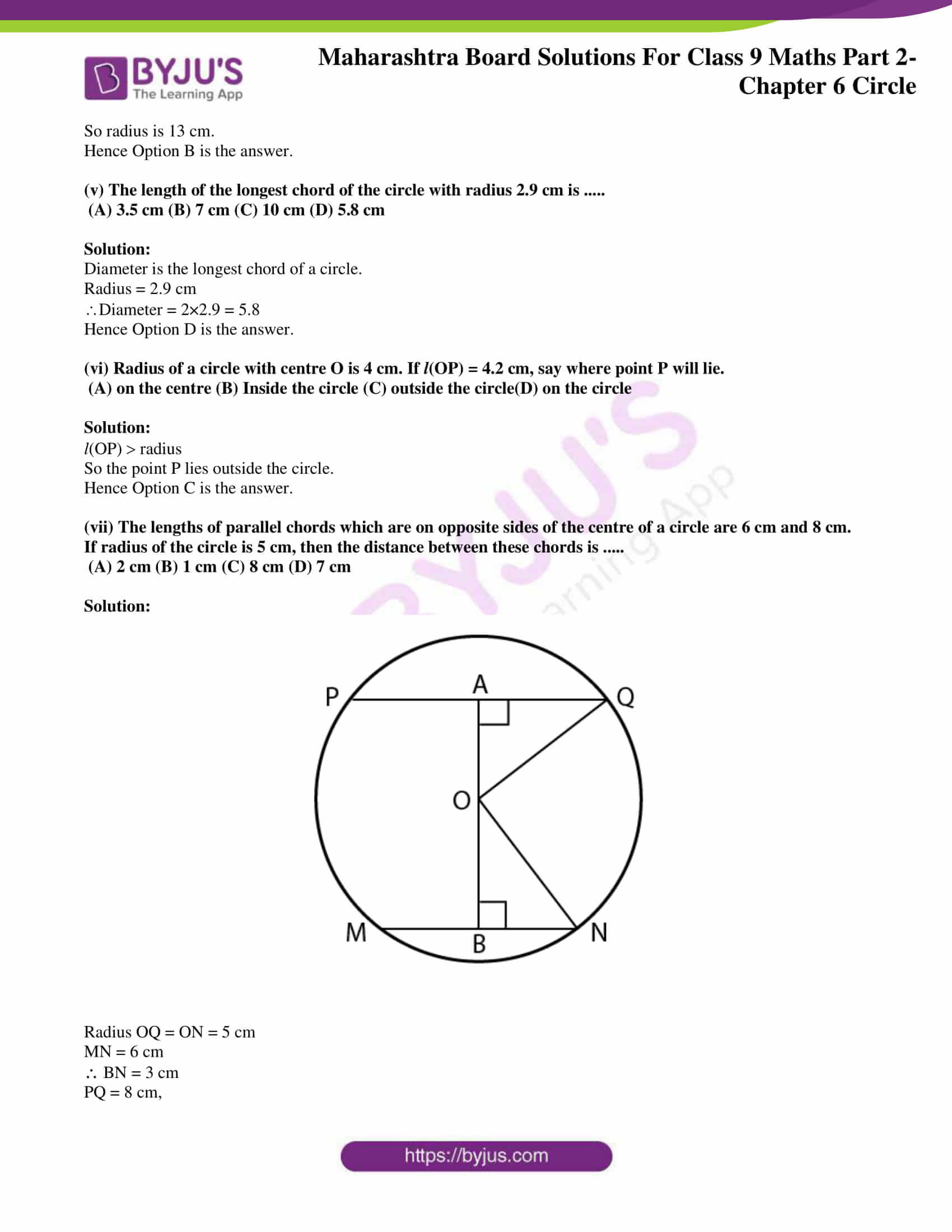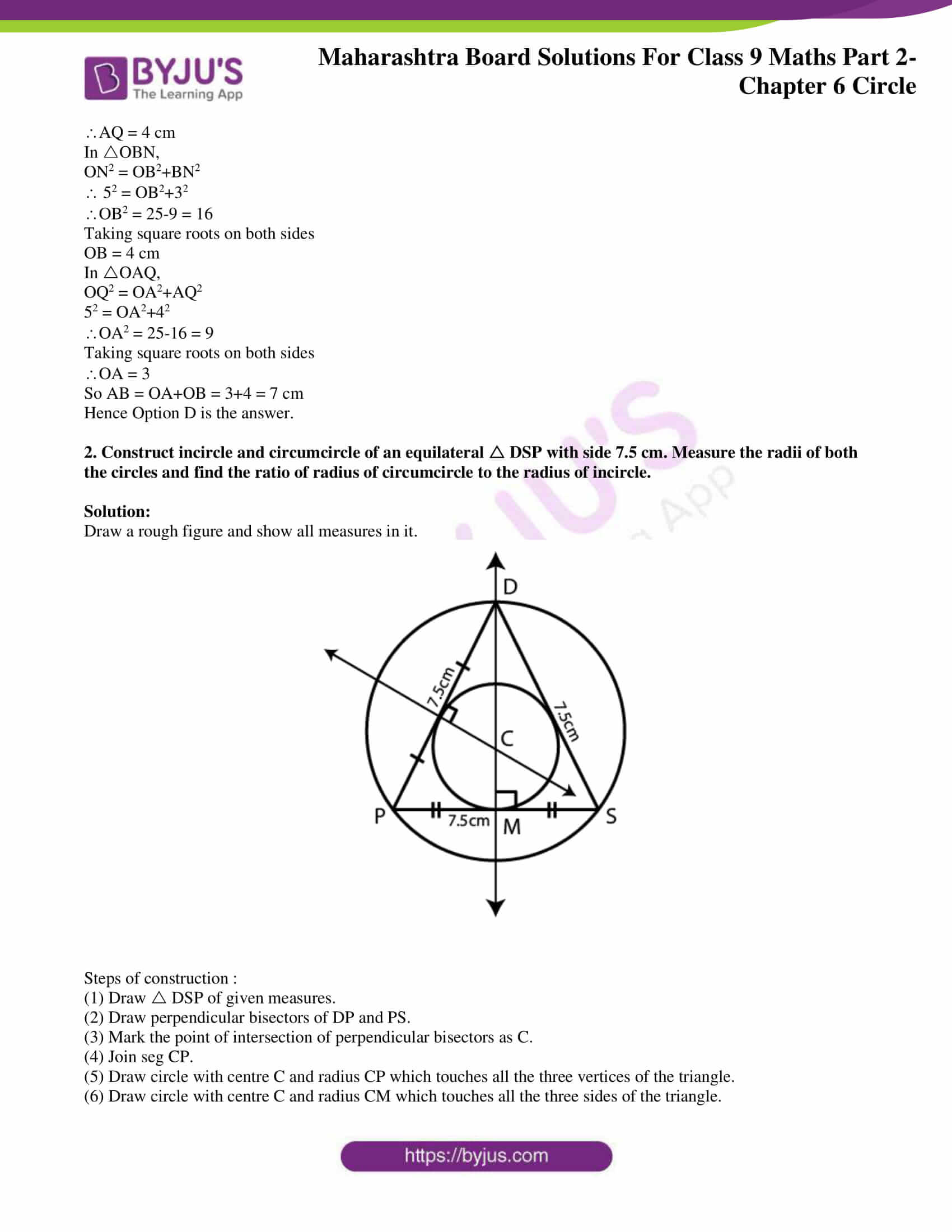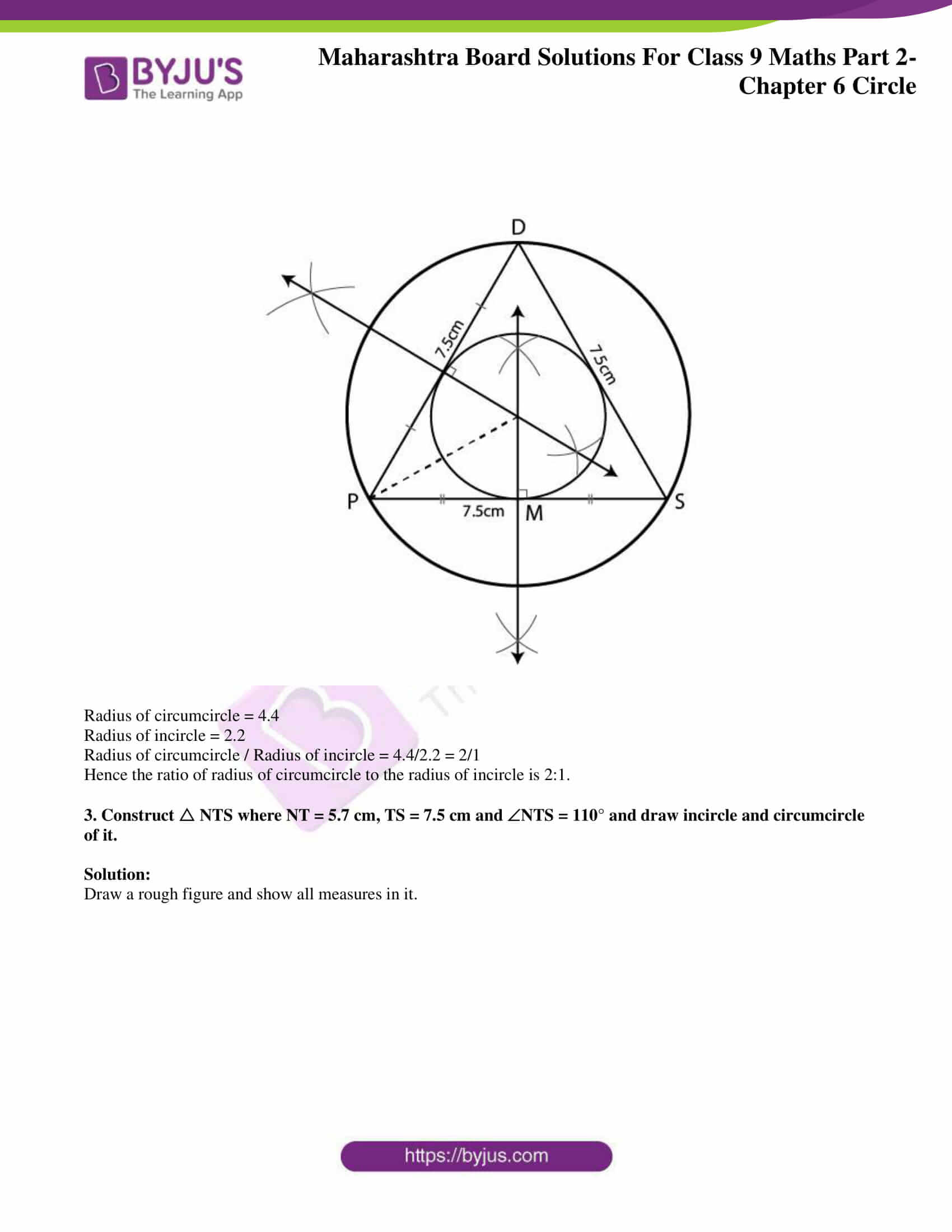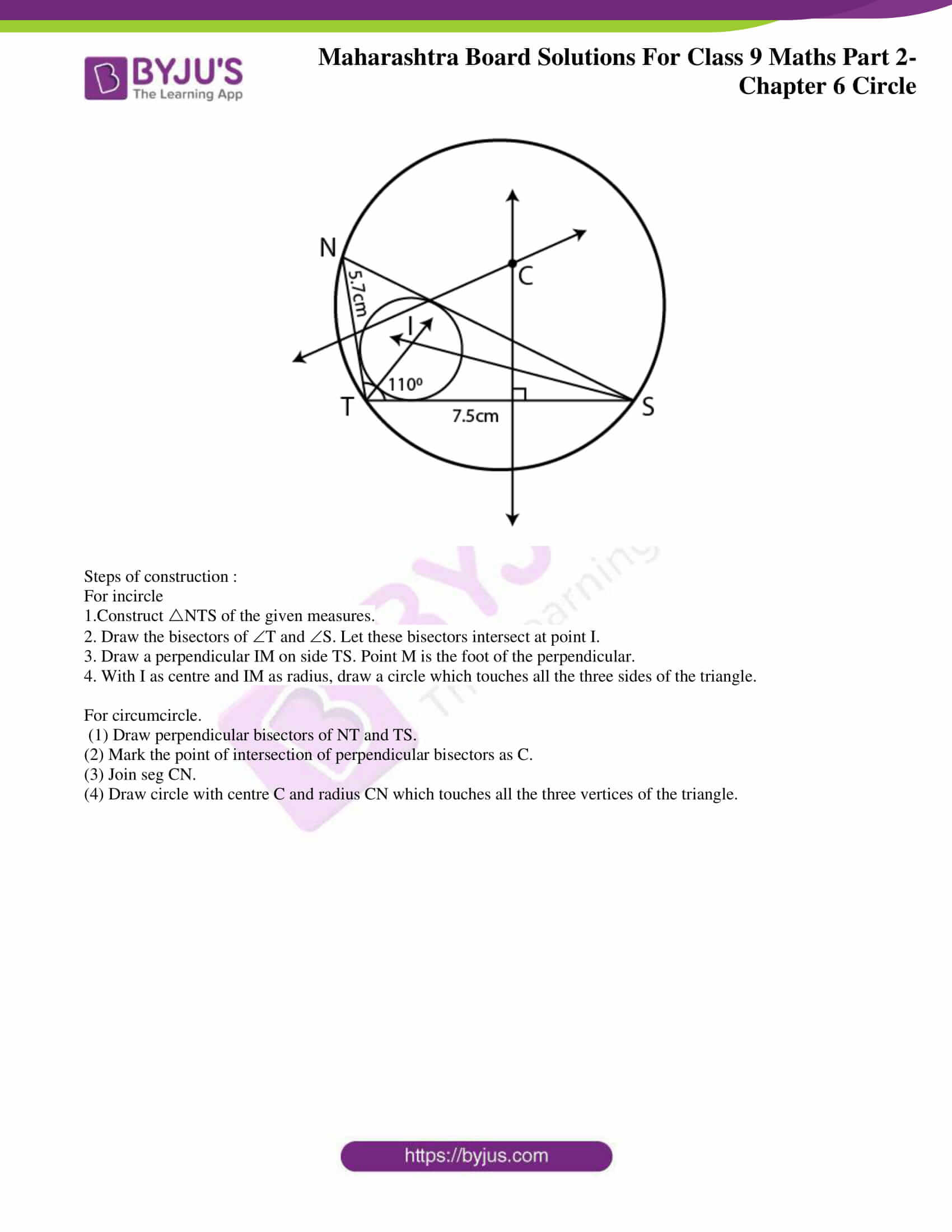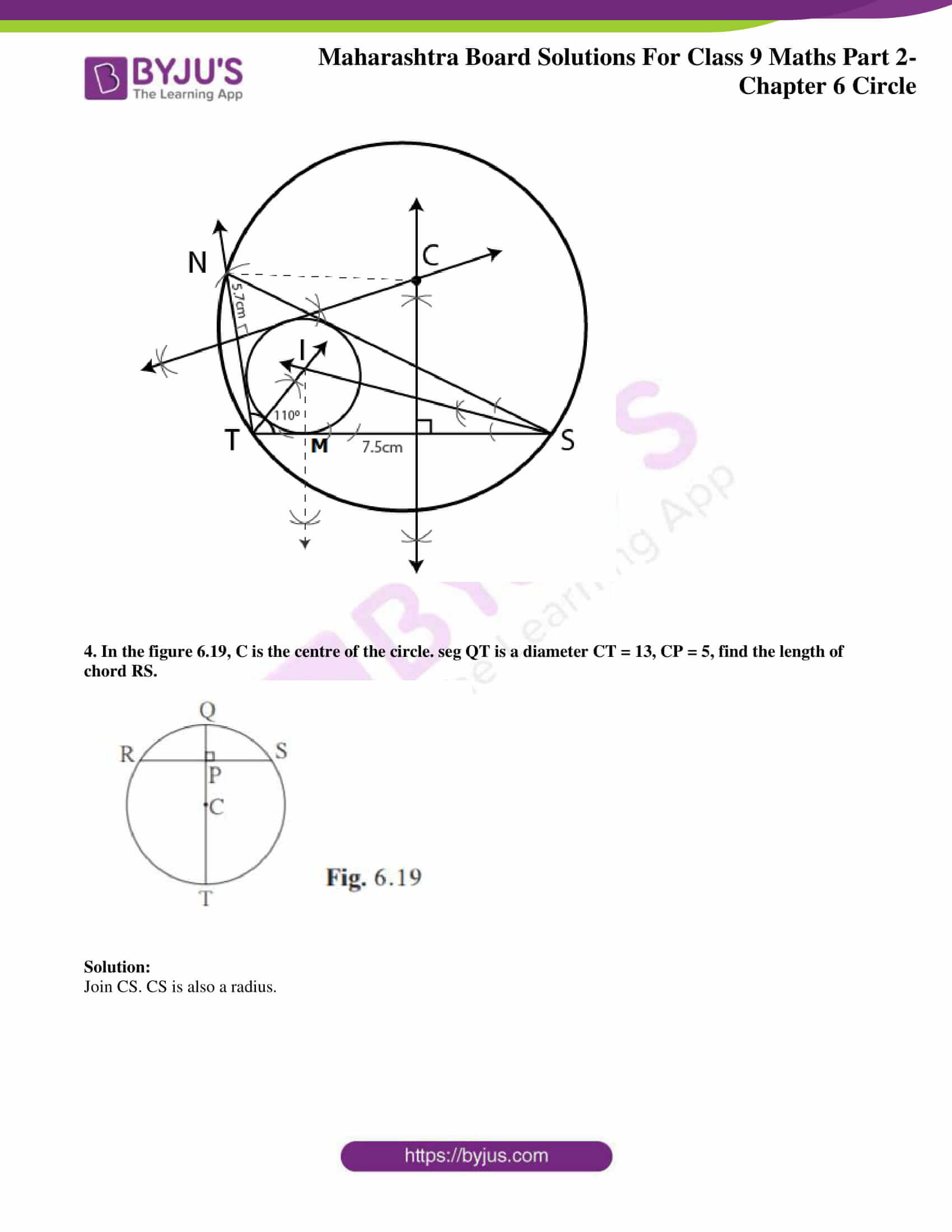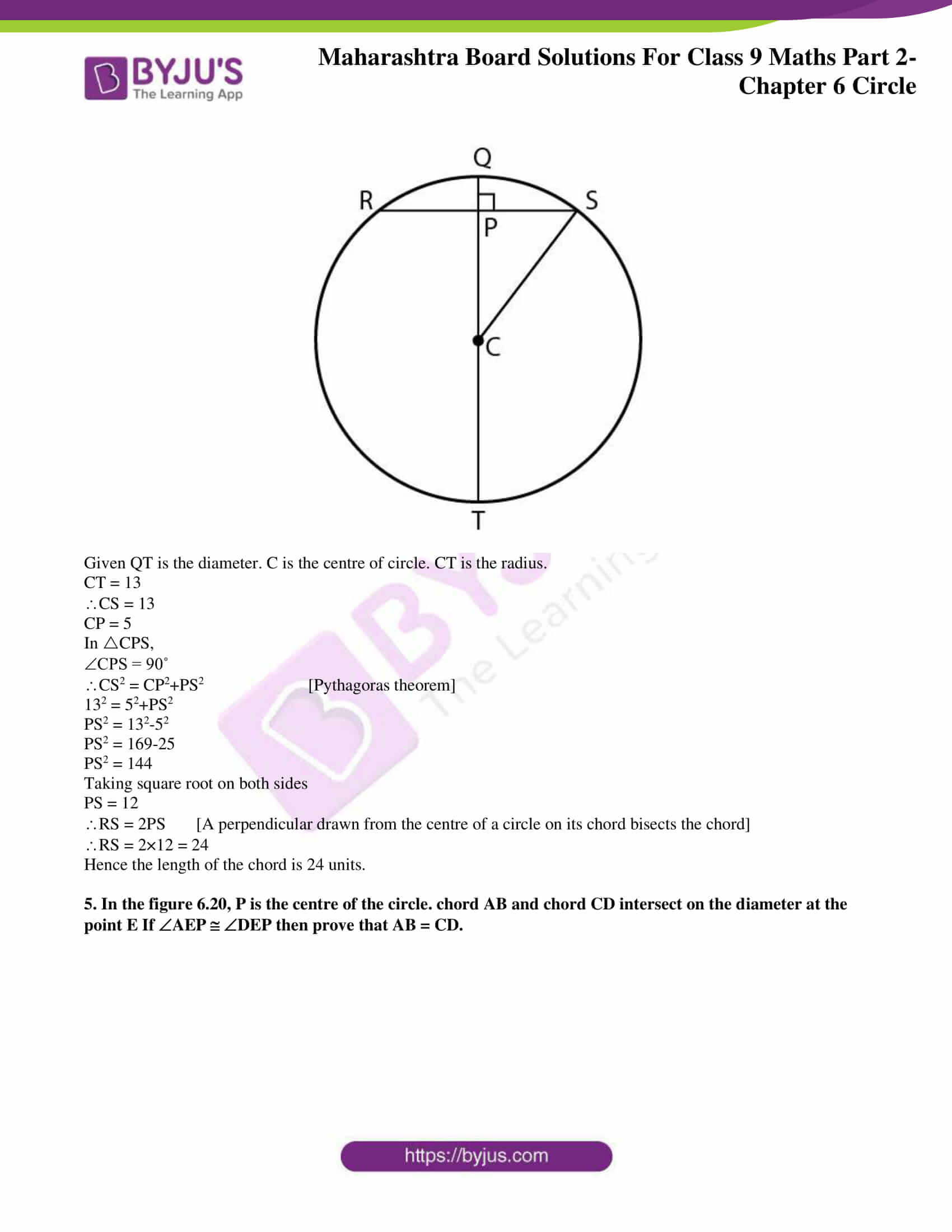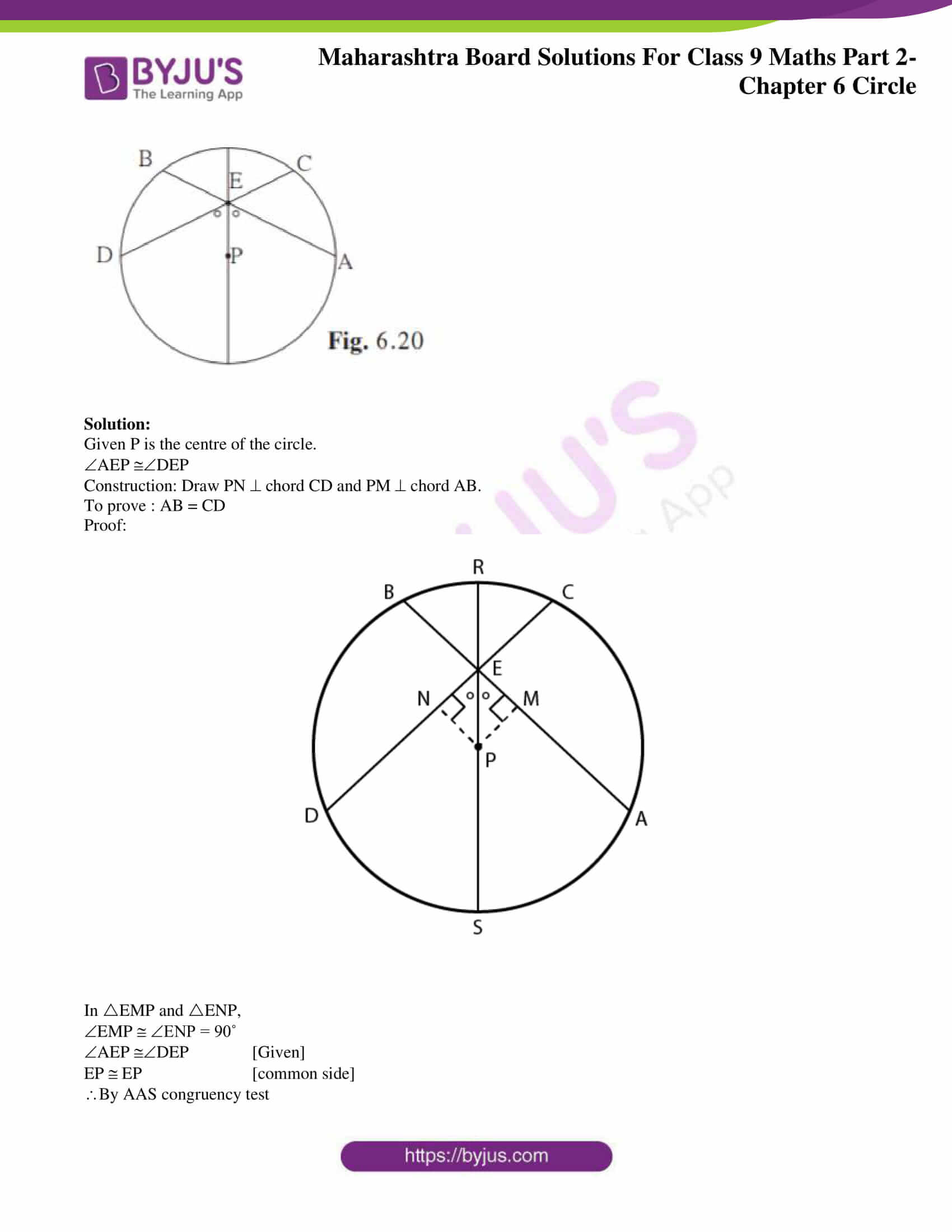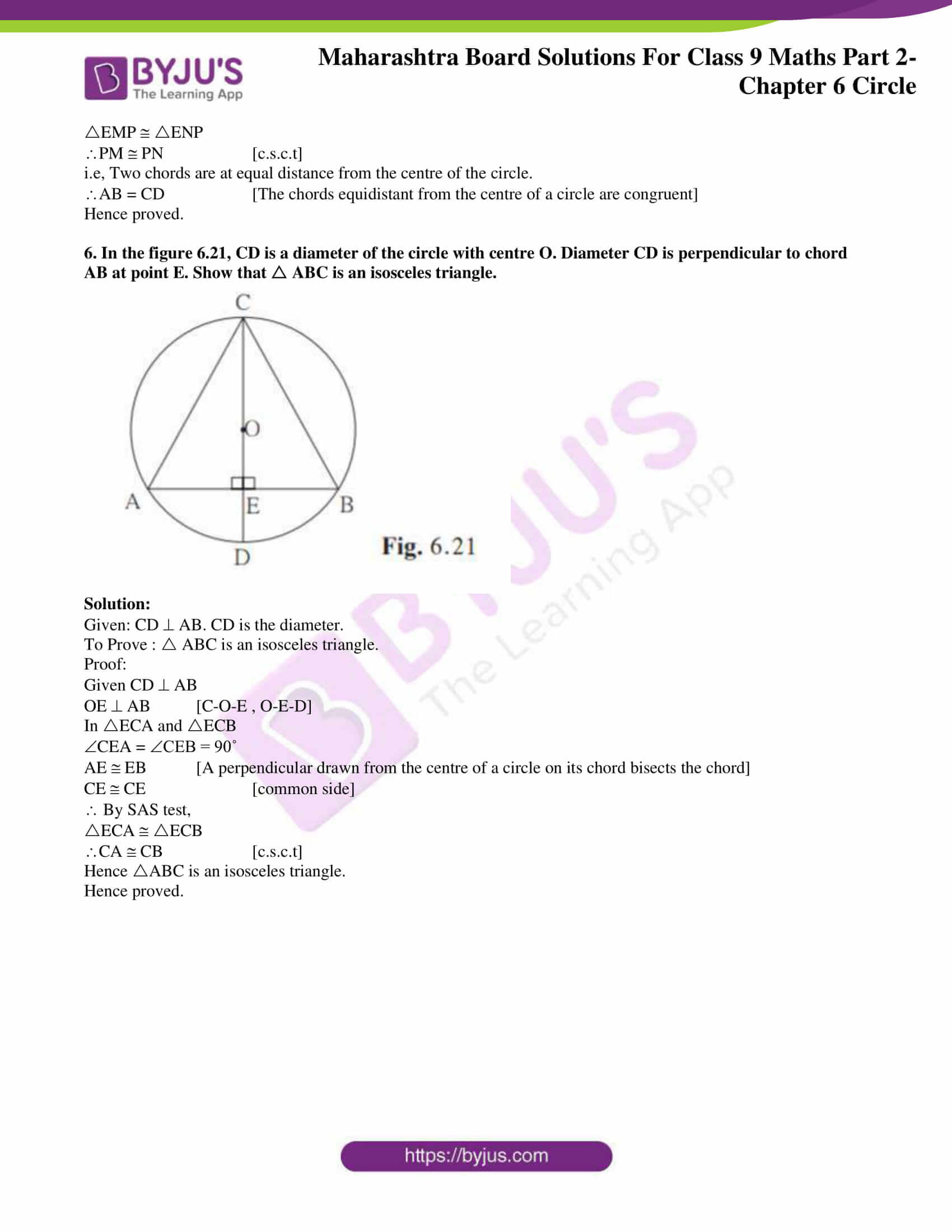### Access answers to Maths MSBSHSE Solutions For Class 9 Part 2 Chapter 6 – Circle

Practice Set 6.1 Page 79

1. Distance of chord AB from the centre of a circle is 8 cm. Length of the chord AB is 12 cm. Find the diameter of the circle.

Solution: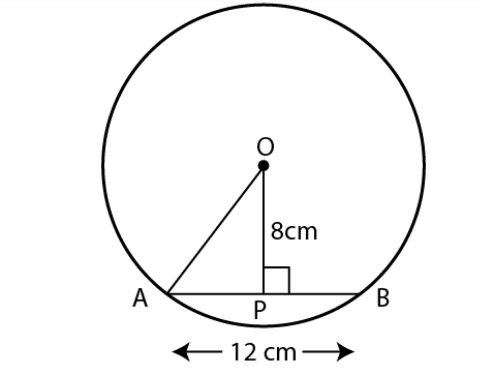Given OP = 8 cm

AB = 12 cm

OP AB

A perpendicular drawn from the centre of a circle on its chord bisects the chord.

AP = ½ AB

AP = 12/2 = 6 cm

OA is the radius of the circle.

In OAP ,

OPA = 90˚

OA2 = OP2+AP2 [Pythagoras theorem]

OA2 = 82+62

OA2 = 64+36= 100

Taking square root on both sides

OA = 10

Radius of circle is 10 cm

Diameter = 2 ×radius = 2×10 = 20 cm

Hence diameter of the circle is 20 cm.

2. Diameter of a circle is 26 cm and length of a chord of the circle is 24 cm. Find the distance of the chord from the centre.

Solution :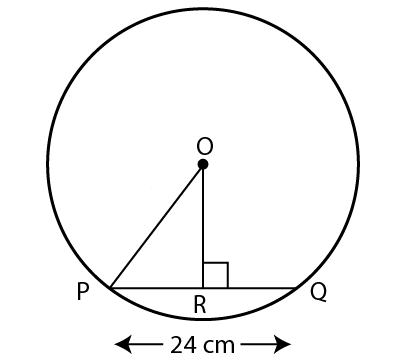Given diameter of the circle = 26 cm

Length of the chord PQ = 24 cm

OP = 26/2 = 13

A perpendicular drawn from the centre of a circle on its chord bisects the chord.

PR = 24/2 = 12 cm

In POR,

ORP = 90˚

OP2 = OR2+PR2 [Pythagoras theorem]

132 = OR2+122

OR2 = 132-122

OR2 = 169-144 = 25

Taking square root on both sides,

OR = 5

Hence distance of the chord from the centre is 5 cm.

3. Radius of a circle is 34 cm and the distance of the chord from the centre is 30 cm, find the length of the chord.

Solution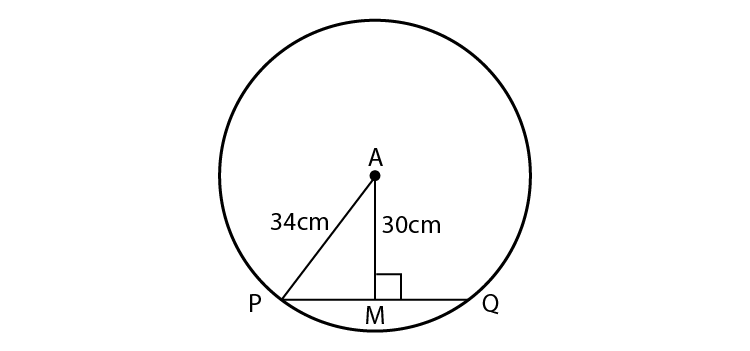Given radius of circle is 34 cm.

AP = 34 cm

Distance of the chord from the centre , AM = 30 cm

In AMP,

AMP = 90˚

AP2= AM2+PM2 [Pythagoras theorem]

342= 302+PM2

PM2 = 342-302

PM2 = 1156-900

PM2 = 256

Taking square root on both sides

PM = 16

A perpendicular drawn from the centre of a circle on its chord bisects the chord.

PQ = 2PM

PQ = 2×16 = 32

Hence the length of the chord is 32 cm.

4. Radius of a circle with centre O is 41 units. Length of a chord PQ is 80 units, find the distance of the chord from the centre of the circle.

Solution: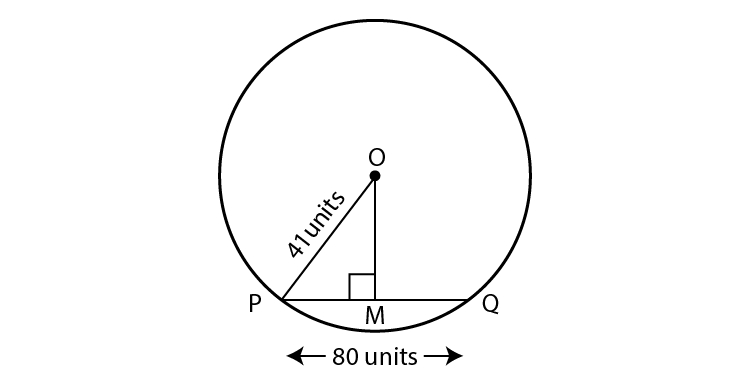Given radius of the circle, OP = 41

Length of the chord, PQ = 80

A perpendicular drawn from the centre of a circle on its chord bisects the chord.

PM = PQ/2

PM = 80/2 = 40

In OMP,

OMP = 90˚

OP2 = OM2+PM2 [Pythagoras theorem]

412 = OM2+402

OM2 = 412-402

OM2 = 1681-1600

OM2 = 81

Taking square root on both sides

OM = 9

Hence the distance of the chord from the centre of the circle is 9 units.

5. In figure 6.9, centre of two circles is O. Chord AB of bigger circle intersects the smaller circle in points P and Q. Show that AP = BQ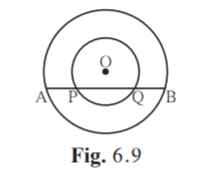Solution:

Draw OX perpendicular to AB.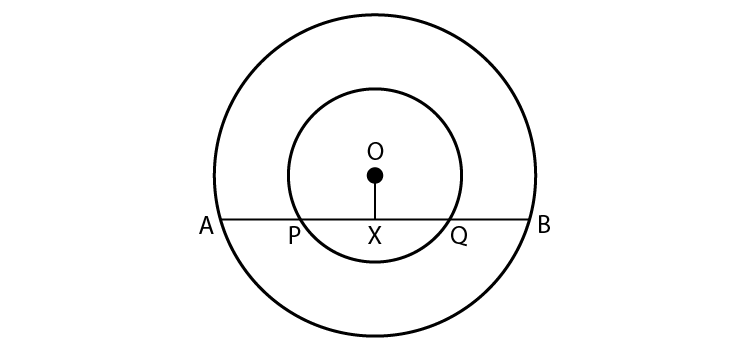Consider smaller circle,

Seg OX seg PQ [A-P-X-Q-B]

A perpendicular drawn from the centre of a circle on its chord bisects the chord.

PX = XQ….(i)

Consider bigger circle,

Seg OX seg AB [A-P-X, X-Q-B]

A perpendicular drawn from the centre of a circle on its chord bisects the chord.

AX = XB

AP+PX = XQ+BQ [A-P-X, X-Q-B]

AP+XQ = XQ+BQ [From (i)]

AP = BQ

Hence proved.

6. Prove that, if a diameter of a circle bisects two chords of the circle then those two chords are parallel to each other.

Solution: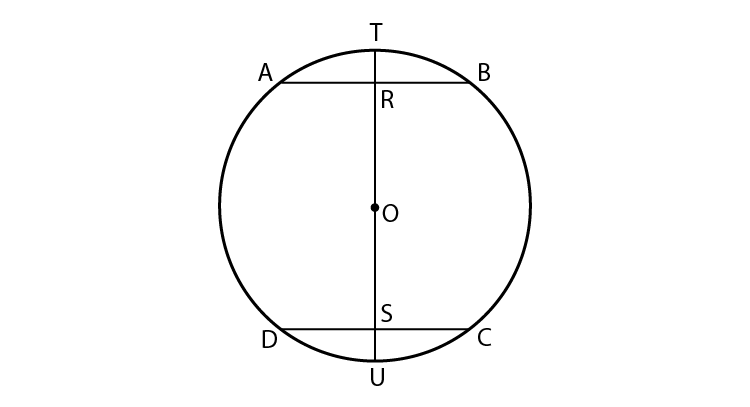Let AB and CD are the two chords.

Let PQ be the diameter passing through centre of circle O.

PQ meets AB at R .

PQ meets DC at S.

When a line passing through centre of circle bisects the chord, then it is perpendicular to the chord.

ARO =CSO = 90˚

For chord AB and CD, PQ act as a transversal.

Also ARO =CSO = 90˚

So alternate interior angles are equal.

Hence we can say chord AB and CD are parallel.

Hence proved.

Practice set 6.2 Page 82

1. Radius of circle is 10 cm. There are two chords of length 16 cm each. What will be the distance of these chords from the centre of the circle ?

Solution: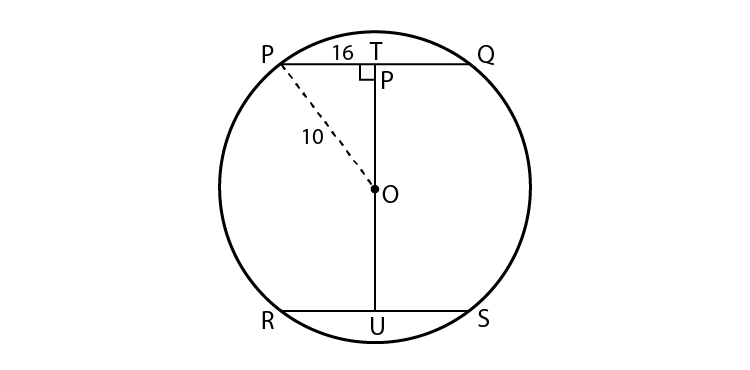Given radius of circle, OP = 10 cm

Length of chord, PQ = 16 cm

Equal chords of a circle are at equal distance from the centre.

OT = OU ….(i)

PT = PQ/2 [A perpendicular drawn from the centre of a circle on its chord bisects the chord]

PT = 16/2 = 8 cm

In PTO,

PTO = 90˚

OP2 = PT2+OT2 [Pythagoras theorem]

102 = 82+OT2

OT2 = 102-82

OT2 = 100-64

OT2 = 36

Taking square root on both sides,

OT = 6

OT = OU [From (i)]

Hence the distance of the chords from the centre of circle is 6 cm .

2. In a circle with radius 13 cm, two equal chords are at a distance of 5 cm from the centre. Find the lengths of the chords.

Solution: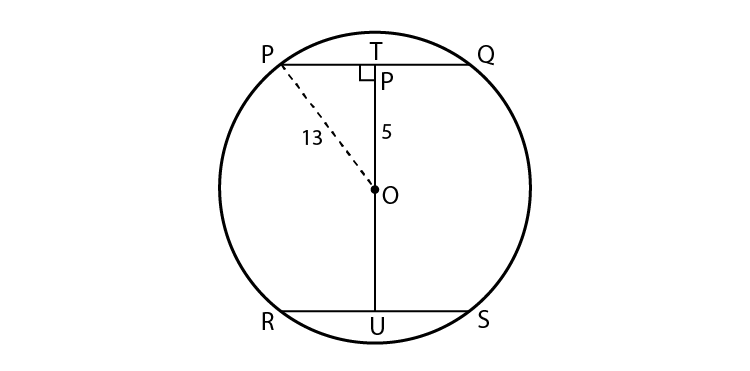Given radius of the circle OP = 13 cm

Distance of the chord from the centre, OT = 5 cm

The chords equidistant from the centre of a circle are congruent.

PQ = RS….(i)

In PTO,

PTO = 90˚

OP2 = PT2+OT2 [Pythagoras theorem]

132 = PT2+52

PT2 = 132-52

PT2 = 169-25

PT2 = 144

Taking square root on both sides,

PT = 12

PT = PQ/2 [A perpendicular drawn from the centre of a circle on its chord bisects the chord]

PQ = 2PT

PQ = 2×12 = 24

RS = 24 [From (i)]

Hence the lengths of the chords are 24 cm.

3. Seg PM and seg PN are congruent chords of a circle with centre C. Show that the ray PC is the bisector of NPM

Solution: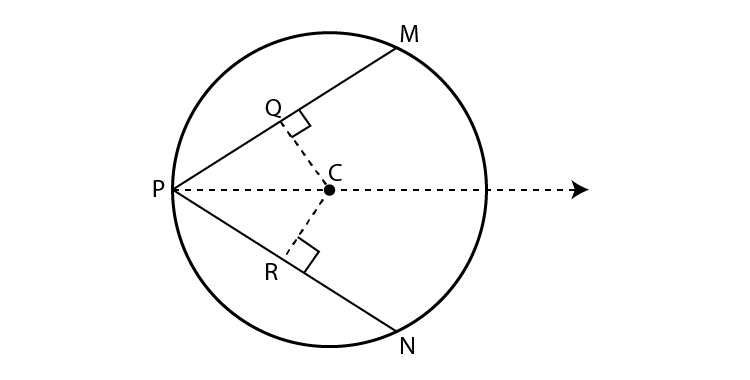Given PM PN

Congruent chords of a circle are equidistant from the centre of the circle.

seg CQ seg CR ….(i)

In CQP and CRP ,

seg CQ seg CR [From (i)]

CQP = CRP = 90˚

seg PC seg PC [Common side]

By hypotenuse side test,

CQP CRP

QPC RPC [c.a.c.t]

MPC NPC [N-R-P, M-Q-P]

PC is the bisector of NPM.

Hence proved.

Practice set 6.3 Page 86

1. Construct ABC such that B =100°, BC = 6.4 cm, C = 50° and construct its incircle.

Solution:

Draw a rough figure and show all measures in it.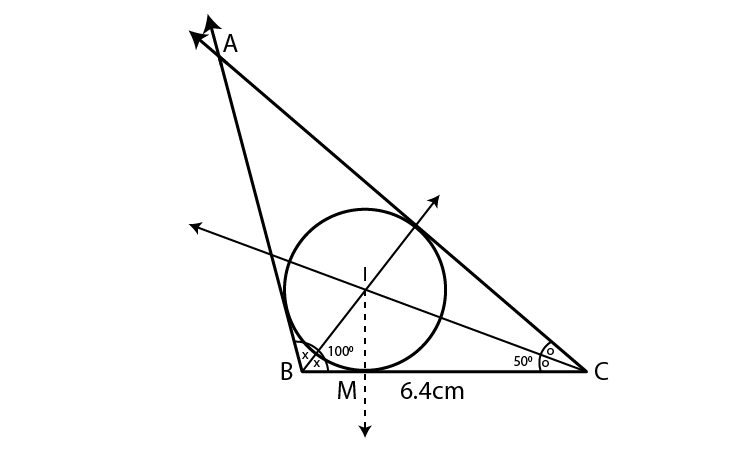Steps of construction :

(1) Draw ABC of given measures.

(2) Draw angle bisectors of B and C

(3) Name the point of intersection of angle bisectors as I.

(4) Draw a perpendicular IM on side BC. Point M is the foot of the perpendicular.

(5) With I as centre and IM as radius, draw a circle which touches all sides of triangle.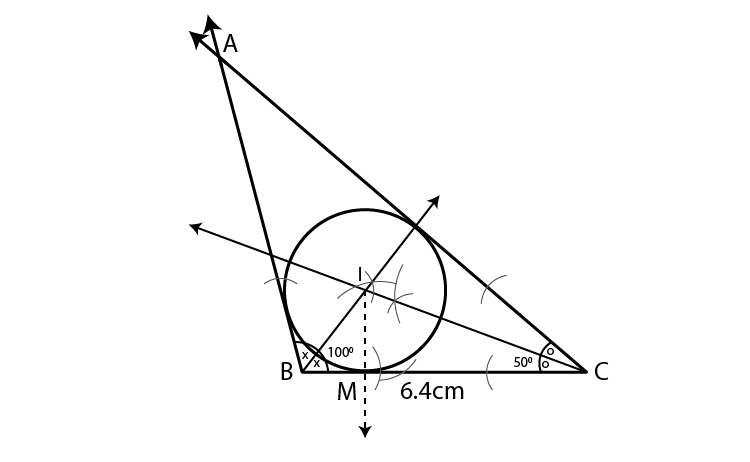2. Construct PQR such that P = 70°, R = 50°, QR = 7.3 cm. and construct its circumcircle.

Solution:

Given P = 70°, R = 50°

P+Q+R = 180˚

70+Q+50 = 180˚

Q = 180-120 = 60˚

Draw a rough figure and show all measures in it.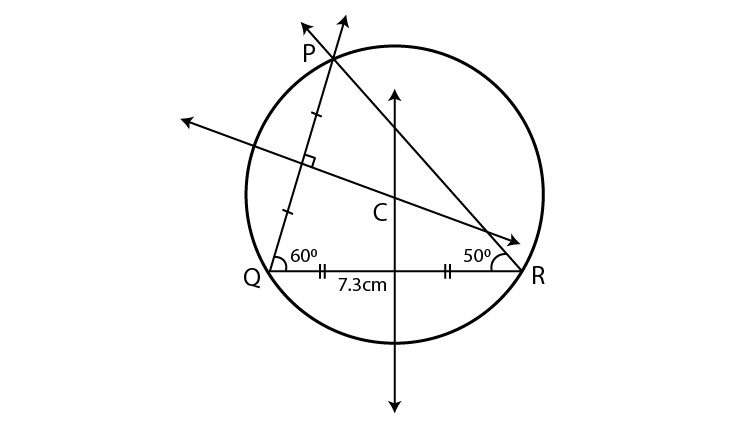Steps of construction :

(1) Draw PQR of given measures.

(2) Draw perpendicular bisectors of PQ and QR.

(3) Name the point of intersection of perpendicular bisectors as C.

(4) Join seg CP.

(5) Draw circle with centre C and radius CP.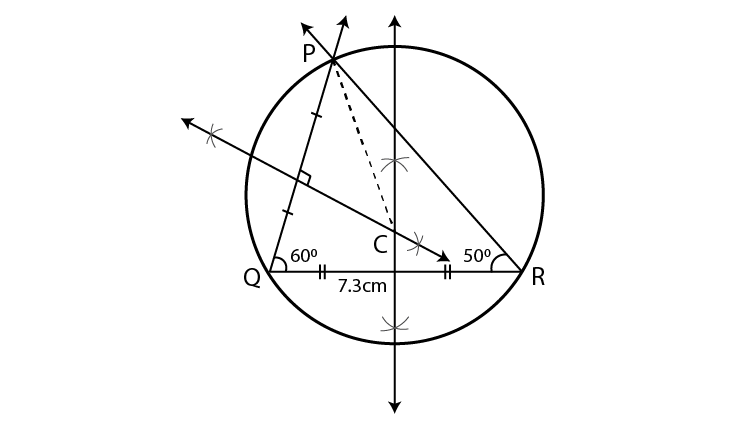3. Construct XYZ such that XY = 6.7 cm, YZ = 5.8 cm, XZ = 6.9 cm. Construct its incircle.

Solution:

Draw a rough figure and show all measures in it.

Steps of construction:

1.Construct XYZ of the given measures.

2. Draw the bisectors of X and Z. Let these bisectors intersect at point I.

3. Draw a perpendicular IM on side XZ. Point M is the foot of the perpendicular.

4. With I as centre and IM as radius, draw a circle which touches all the three sides of the triangle.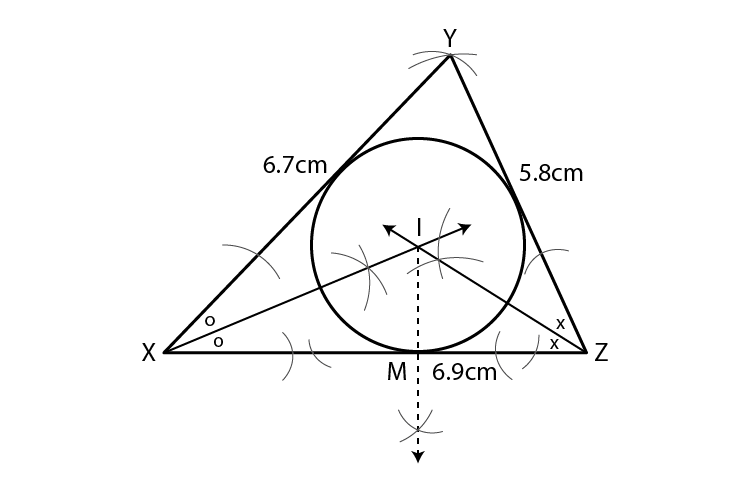4. In LMN, LM = 7.2 cm, M = 105°, MN = 6.4 cm, then draw LMN and construct its circumcircle.

Solution:

Draw a rough figure and show all measures in it.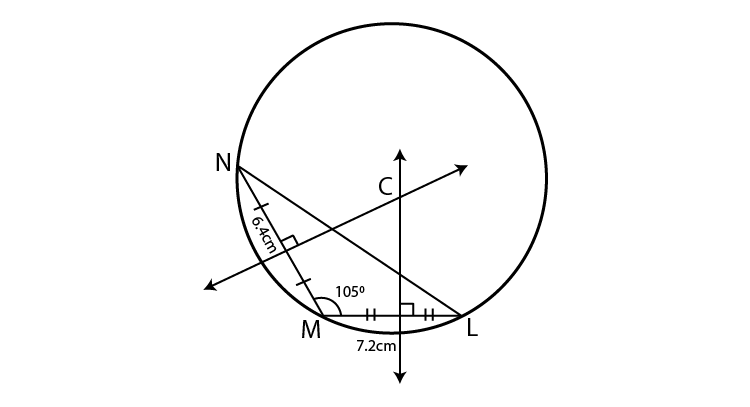Steps of construction :

(1) Draw LMN of given measures.

(2) Draw perpendicular bisectors of ML and MN.

(3) Name the point of intersection of perpendicular bisectors as C.

(4) Join seg CM.

(5) Draw circle with centre C and radius CM which touches all the three vertices of the triangle.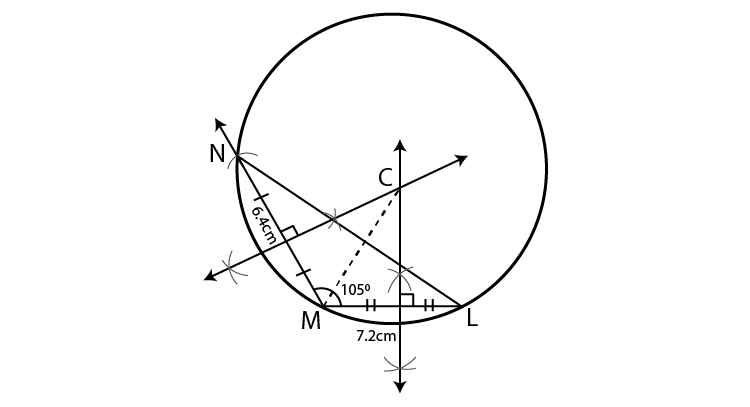5. Construct DEF such that DE = EF = 6 cm, F = 45° and construct its circumcircle.

Solution:

Draw a rough figure and show all measures in it.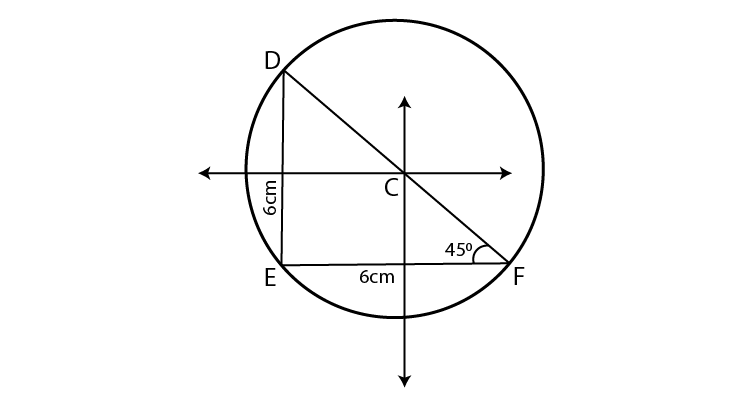Steps of construction :

(1) Draw DEF of given measures.

(2) Draw perpendicular bisectors of DE and EF.

(3) Name the point of intersection of perpendicular bisectors as C.

(4) Join seg CE.

(5) Draw circle with centre C and radius CE which touches all the three vertices of the triangle.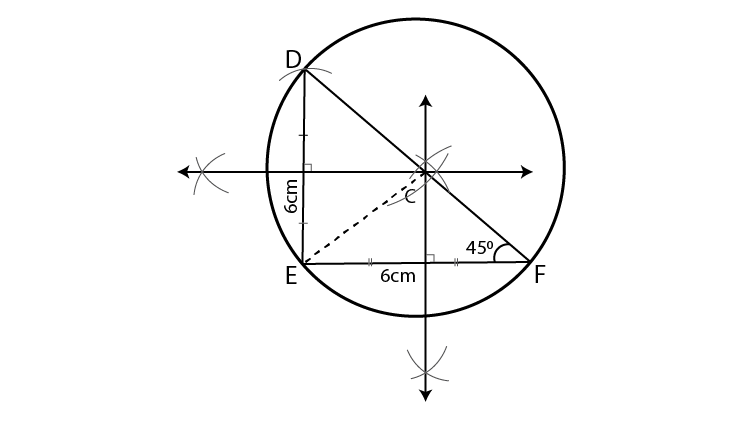Problem Set 6 Page 86

1. Choose correct alternative answer and fill in the blanks.

(i) Radius of a circle is 10 cm and distance of a chord from the centre is 6 cm.

Hence the length of the chord is ………

(A) 16 cm (B) 8 cm (C) 12 cm (D) 32 cm

Solution: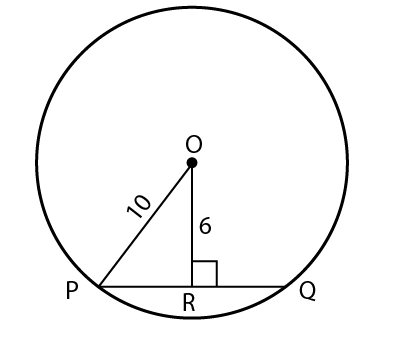Distance of chord from the circle, OR = 6

In OPR ,

ORP = 90˚

OP2= OR2+PR2 [Pythagoras theorem]

102= 62+PR2

PR2 = 102-62

PR2 = 100-36

PR2 = 64

Taking square root on both sides

PR = 8

A perpendicular drawn from the centre of a circle on its chord bisects the chord.

PQ = 2PR

PQ = 2×8 = 16

Hence the length of the chord is 16 cm.

Hence Option A is the answer.

(ii) The point of concurrence of all angle bisectors of a triangle is called the ……

(A) centroid (B) circumcentre (C) incentre (D) orthocentre

Solution:

The point of concurrence of all angle bisectors of a triangle is called the incentre.

Hence Option C is the answer.

(iii) The circle which passes through all the vertices of a triangle is called …..

(A) circumcircle (B) incircle (C) congruent circle (D) concentric circle

Solution:

The circle which passes through all the vertices of a triangle is called circumcircle.

Hence Option A is the answer.

(iv) Length of a chord of a circle is 24 cm. If distance of the chord from the centre is 5 cm, then the radius of that circle is ….

(A) 12 cm (B) 13 cm (C) 14 cm (D) 15 cm

Solution: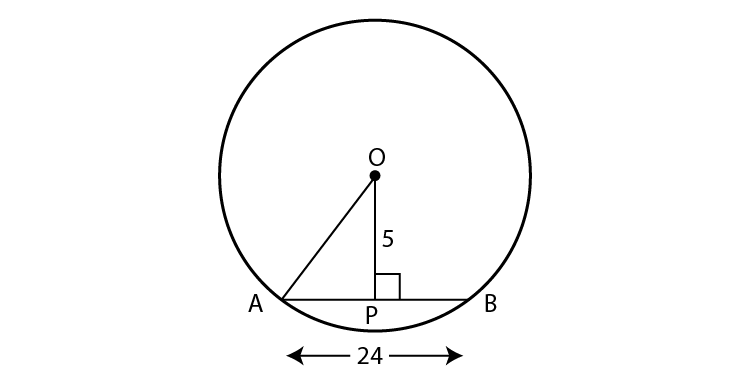Given length of the chord, AB = 24 cm

Distance of the chord from the circle, OP = 5 cm

AP = AB/2 [A perpendicular drawn from the centre of a circle on its chord bisects the chord]

AP = 24/2 = 12 cm

In OAP ,

OPA = 90˚

OA2= OP2+AP2 [Pythagoras theorem]

OA2= 52+122

OA2 = 25+144

OA2 = 169

Taking square root on both sides

OA = 13

Hence Option B is the answer.

(v) The length of the longest chord of the circle with radius 2.9 cm is …..

(A) 3.5 cm (B) 7 cm (C) 10 cm (D) 5.8 cm

Solution:

Diameter is the longest chord of a circle.

Diameter = 2×2.9 = 5.8

Hence Option D is the answer.

(vi) Radius of a circle with centre O is 4 cm. If l(OP) = 4.2 cm, say where point P will lie.

(A) on the centre (B) Inside the circle (C) outside the circle(D) on the circle

Solution:

So the point P lies outside the circle.

Hence Option C is the answer.

(vii) The lengths of parallel chords which are on opposite sides of the centre of a circle are 6 cm and 8 cm. If radius of the circle is 5 cm, then the distance between these chords is …..

(A) 2 cm (B) 1 cm (C) 8 cm (D) 7 cm

Solution: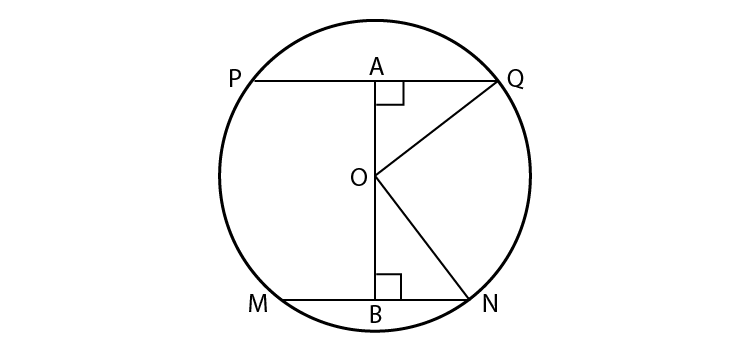Radius OQ = ON = 5 cm

MN = 6 cm

BN = 3 cm

PQ = 8 cm,

AQ = 4 cm

In OBN,

ON2 = OB2+BN2

52 = OB2+32

OB2 = 25-9 = 16

Taking square roots on both sides

OB = 4 cm

In OAQ,

OQ2 = OA2+AQ2

52 = OA2+42

OA2 = 25-16 = 9

Taking square roots on both sides

OA = 3

So AB = OA+OB = 3+4 = 7 cm

Hence Option D is the answer.

2. Construct incircle and circumcircle of an equilateral DSP with side 7.5 cm. Measure the radii of both the circles and find the ratio of radius of circumcircle to the radius of incircle.

Solution:

Draw a rough figure and show all measures in it.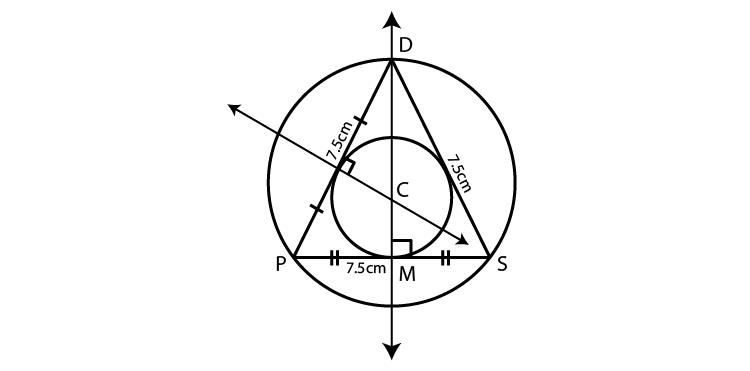Steps of construction :

(1) Draw DSP of given measures.

(2) Draw perpendicular bisectors of DP and PS.

(3) Mark the point of intersection of perpendicular bisectors as C.

(4) Join seg CP.

(5) Draw circle with centre C and radius CP which touches all the three vertices of the triangle.

(6) Draw circle with centre C and radius CM which touches all the three sides of the triangle.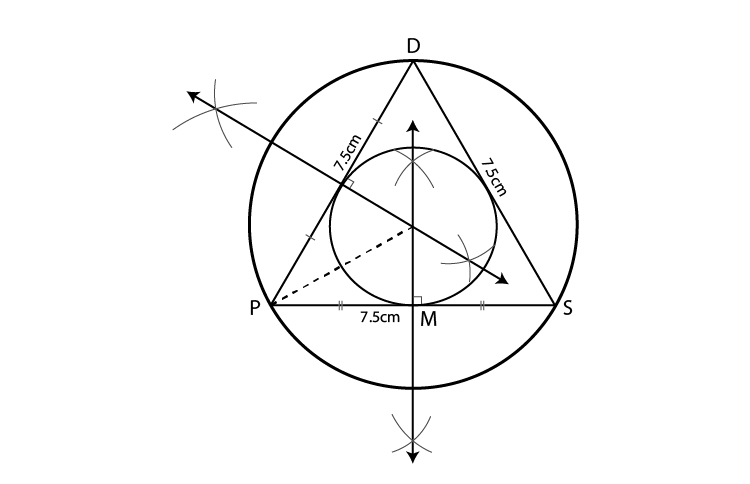Hence the ratio of radius of circumcircle to the radius of incircle is 2:1.

3. Construct NTS where NT = 5.7 cm, TS = 7.5 cm and NTS = 110° and draw incircle and circumcircle of it.

Solution:

Draw a rough figure and show all measures in it.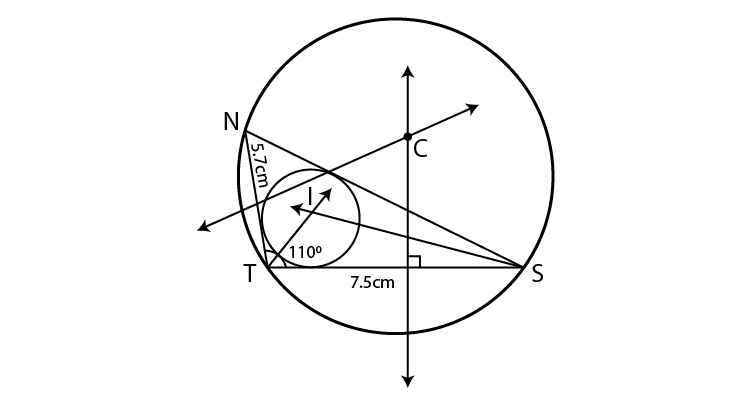Steps of construction :

For incircle

1.Construct NTS of the given measures.

2. Draw the bisectors of T and S. Let these bisectors intersect at point I.

3. Draw a perpendicular IM on side TS. Point M is the foot of the perpendicular.

4. With I as centre and IM as radius, draw a circle which touches all the three sides of the triangle.

For circumcircle.

(1) Draw perpendicular bisectors of NT and TS.

(2) Mark the point of intersection of perpendicular bisectors as C.

(3) Join seg CN.

(4) Draw circle with centre C and radius CN which touches all the three vertices of the triangle.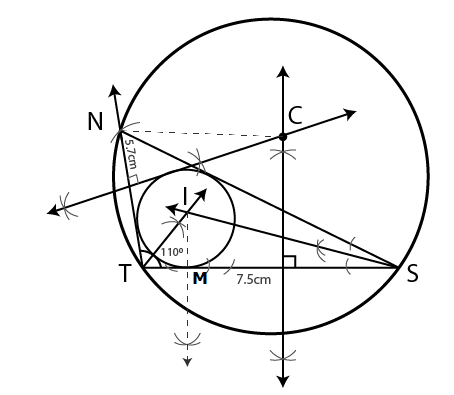4. In the figure 6.19, C is the centre of the circle. seg QT is a diameter CT = 13, CP = 5, find the length of chord RS.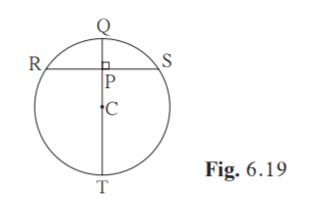Solution:

Join CS. CS is also a radius.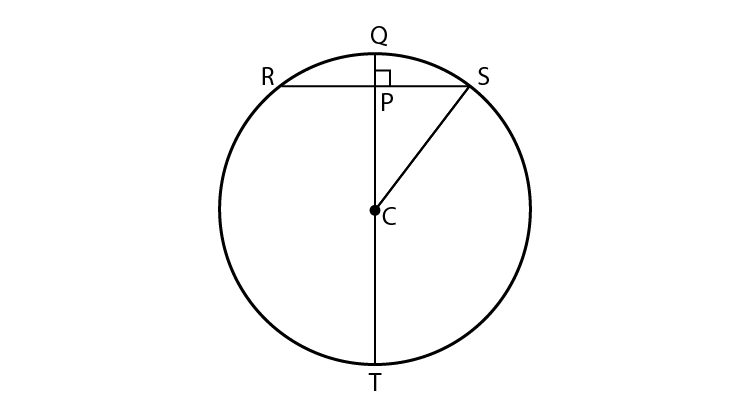Given QT is the diameter. C is the centre of circle. CT is the radius.

CT = 13

CS = 13

CP = 5

In CPS,

CPS = 90˚

CS2 = CP2+PS2 [Pythagoras theorem]

132 = 52+PS2

PS2 = 132-52

PS2 = 169-25

PS2 = 144

Taking square root on both sides

PS = 12

RS = 2PS [A perpendicular drawn from the centre of a circle on its chord bisects the chord]

RS = 2×12 = 24

Hence the length of the chord is 24 units.

5. In the figure 6.20, P is the centre of the circle. chord AB and chord CD intersect on the diameter at the point E If AEP DEP then prove that AB = CD.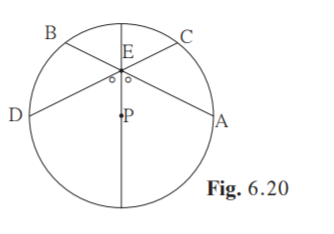Solution:

Given P is the centre of the circle.

AEP DEP

Construction: Draw PN chord CD and PM chord AB.

To prove : AB = CD

Proof: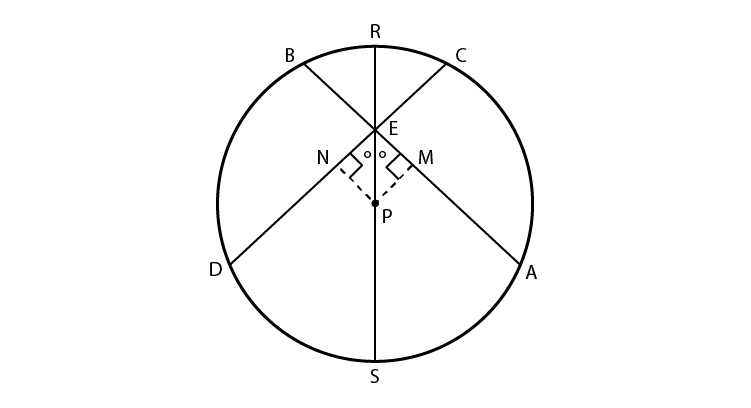In EMP and ENP,

EMP ENP = 90˚

AEP DEP [Given]

EP EP [common side]

By AAS congruency test

EMP ENP

PM PN [c.s.c.t]

i.e, Two chords are at equal distance from the centre of the circle.

AB = CD [The chords equidistant from the centre of a circle are congruent]

Hence proved.

6. In the figure 6.21, CD is a diameter of the circle with centre O. Diameter CD is perpendicular to chord AB at point E. Show that ABC is an isosceles triangle.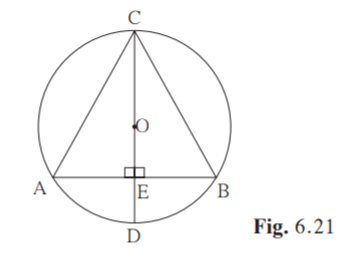Solution:

Given: CD AB. CD is the diameter.

To Prove : ABC is an isosceles triangle.

Proof:

Given CD AB

OE AB [C-O-E , O-E-D]

In ECA and ECB

CEA = CEB = 90˚

AE EB [A perpendicular drawn from the centre of a circle on its chord bisects the chord]

CE CE [common side]

By SAS test,

ECA ECB

CA CB [c.s.c.t]

Hence ABC is an isosceles triangle.

Hence proved.

This chapter deals with properties of chord and relation between congruent chords of a circle and their distances from the centre. The construction of incircle and circumcircle is explained in this chapter. Visit BYJU’S to get more information on concepts and a greater level of understanding Circle and its solutions.

## Frequently Asked Questions on Maharashtra State Board Solutions for Class 9 Maths Part 2 Chapter 6 Circle

### Do these Maharashtra State Board Class 9 Maths Part 2 Chapter 6 Solutions help to prepare for the exams?

Yes, these solutions are very useful. Students are highly encouraged to practise these solutions after revising the subjects, as they set the basis for the questions that could get asked often in the board exams.

### Can these Maharashtra State Board Class 9 Maths Part 2 Chapter 6 Solutions be downloaded?

Yes, it is possible to download the solutions. The solutions are given as a scrollable PDF, and we have also mentioned the clickable link for the students to access. Meanwhile, the questions and the solutions  are also made available online on our webpage.

### How to make the most of these Maharashtra State Board Class 9 Maths Part 2 Chapter 6 Solutions?

Students can time themselves while answering the questions as it helps to help them manage the time better during exams. After solving the questions referring to the solutions will help to identify mistakes and avoid them for the exams. This is also a good way to self analyse one’s exam preparations.